# US6178049B1 - Zoom lens - Google Patents

## Info

Publication number
US6178049B1
US6178049B1 US09/227,343 US22734399A US6178049B1 US 6178049 B1 US6178049 B1 US 6178049B1 US 22734399 A US22734399 A US 22734399A US 6178049 B1 US6178049 B1 US 6178049B1
Authority
US
United States
Prior art keywords
lens
lens unit
positive
image
numerical
Prior art date
Legal status (The legal status is an assumption and is not a legal conclusion. Google has not performed a legal analysis and makes no representation as to the accuracy of the status listed.)
Application number
US09/227,343
Inventor
Hitoshi Mukaiya
Akihisa Horiuchi
Current Assignee (The listed assignees may be inaccurate. Google has not performed a legal analysis and makes no representation or warranty as to the accuracy of the list.)
Canon Inc
Original Assignee
Canon Inc
Priority date (The priority date is an assumption and is not a legal conclusion. Google has not performed a legal analysis and makes no representation as to the accuracy of the date listed.)
Filing date
Publication date
Priority to JP8-086616 priority Critical
Priority to JP08661696A priority patent/JP3696966B2/en
Priority to JP8661896A priority patent/JPH09281391A/en
Priority to JP8-086618 priority
Priority to JP14368596A priority patent/JP3679502B2/en
Priority to JP8-143685 priority
Priority to US08/831,730 priority patent/US6226130B1/en
Application filed by Canon Inc filed Critical Canon Inc
Priority to US09/227,343 priority patent/US6178049B1/en
Application granted granted Critical
Publication of US6178049B1 publication Critical patent/US6178049B1/en
Anticipated expiration legal-status Critical
Expired - Lifetime legal-status Critical Current

• 230000005499 meniscus Effects 0.000 claims description 7
• 238000000926 separation method Methods 0.000 abstract description 34
• 230000003287 optical Effects 0.000 abstract description 17
• 230000000694 effects Effects 0.000 abstract description 6
• 230000004075 alteration Effects 0.000 description 59
• 238000010586 diagram Methods 0.000 description 15
• 239000011521 glass Substances 0.000 description 12
• 238000004904 shortening Methods 0.000 description 5
• 230000001629 suppression Effects 0.000 description 4
• 238000010276 construction Methods 0.000 description 3
• 210000001747 Pupil Anatomy 0.000 description 2
• 235000020127 ayran Nutrition 0.000 description 2
• 239000000470 constituent Substances 0.000 description 2
• 239000007787 solid Substances 0.000 description 2
• 230000003595 spectral Effects 0.000 description 2
• 201000009310 astigmatism Diseases 0.000 description 1
• 230000003247 decreasing Effects 0.000 description 1
• 230000000087 stabilizing Effects 0.000 description 1

## Images

•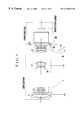•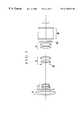•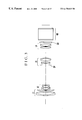•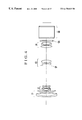•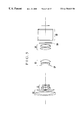•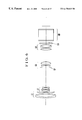•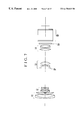•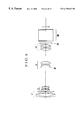•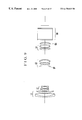•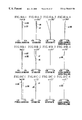•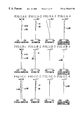•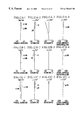•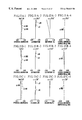•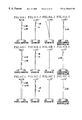•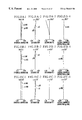•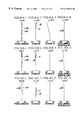•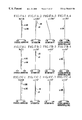•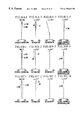•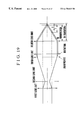•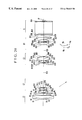•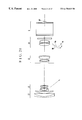•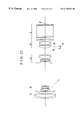•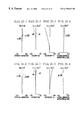••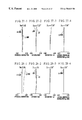•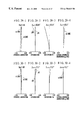•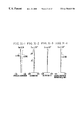## Classifications

• GPHYSICS
• G02OPTICS
• G02BOPTICAL ELEMENTS, SYSTEMS, OR APPARATUS
• G02B15/00Optical objectives with means for varying the magnification
• G02B15/14Optical objectives with means for varying the magnification by axial movement of one or more lenses or groups of lenses relative to the image plane for continuously varying the equivalent focal length of the objective
• G02B15/144Optical objectives with means for varying the magnification by axial movement of one or more lenses or groups of lenses relative to the image plane for continuously varying the equivalent focal length of the objective having four groups only
• G02B15/1441Optical objectives with means for varying the magnification by axial movement of one or more lenses or groups of lenses relative to the image plane for continuously varying the equivalent focal length of the objective having four groups only the first group being positive
• G02B15/144113Optical objectives with means for varying the magnification by axial movement of one or more lenses or groups of lenses relative to the image plane for continuously varying the equivalent focal length of the objective having four groups only the first group being positive arranged +-++

## Abstract

A zoom lens of the rear focus type is disclosed, whose back focal distance is increased to accommodate a three-color separation prism and other optical elements, comprising, in order from an object side to an image side, a first lens unit of positive refractive power, a second lens unit of negative refractive power, a third lens unit of positive refractive power and a fourth lens unit of positive refractive power, the second lens unit and the fourth lens unit being moved to effect zooming and the fourth lens unit being moved to effect focusing, wherein the third lens unit has a negative lens disposed closest to the object side and having a concave surface facing the object side and has a positive lens disposed closest to the image side, and a lens surface on the image side of the positive lens has a refractive power which is stronger than that of a lens surface on the object side of the positive lens.

## Description

This is a division of application Ser. No. 08/831,730 filed Apr. 1, 1997.
BACKGROUND OF THE INVENTION
1. Field of the Invention
This invention relates to zoom lenses of the rear focus type and, more particularly, to zoom lenses of the rear focus type with a color separation prism in the space between the rear vertex and the CCD. Still more particularly, this invention relates to high range, large relative aperture zoom lenses of the rear focus type which provide, despite securing of such a long back focal distance, increase of the zoom ratio while still permitting the diameter of the front lens members to be minimized.
2. Description of Related Art
Recently, home video cameras of ever smaller size and lighter weight have been developed. Along with this development, a remarkable advance is seen even in decreasing the bulk and size of its taking zoom lens. In particular, efforts are being devoted to shortening of the total length of the entire lens system, reduction of the diameter of the front lens members and simplification of the form and the construction and arrangement of the constituent parts.
To attain these ends, one means is to move a lens unit other than the front or first lens unit to effect focusing. Such a zoom lens is known as the so-called “rear focus type”.
In general, the rear focus type of zoom lens has many advantages over the type which performs focusing by moving the front lens unit. For example, it becomes easier to improve the compact form of the entire lens system. Close-up photography, particularly supershort focusing, becomes possible. Further, since the focusing lens unit is of small size and light weight, because the required driving torque for moving the focusing lens unit is reduced, rapid focus adjustment can be carried out.
Such a rear focus type of zoom lens is disclosed in, for example, Japanese Laid-Open Patent Applications Nos. Sho 62-206516, Sho 62-215225 and Sho 62-24213, in which the zoom lens comprises, in order from an object side, a positive first lens unit, a negative second lens unit, a positive third lens unit and a positive fourth lens unit, the second lens unit being axially moved to vary the focal length, and the fourth lens unit being axially moved to compensate for the image shift with zooming and also to effect focusing.
Also, in Japanese Laid-Open Patent Applications Nos. Hei 4-43311, Hei 4-153615, Hei 5-19165, Hei 5-27167 and Hei 5-60973, there are disclosed examples of the fourth lens unit consisting of one or two positive lenses. Also, in Japanese Laid-Open Patent Application No. Hei 5-60974, there is disclosed a zoom lens whose fourth lens unit is constructed with positive and negative lenses, totaling two lenses.
Further, in Japanese Laid-Open Patent Applications Nos. Sho 55-62419, Sho 62-24213, Sho 62-215225, Sho 56-114920, Hei 3-200113, Hei 4-242707, Hei 4-343313 and Hei 5-297275, there are disclosed, in their embodiments, zoom lenses in which the third and fourth lens units each are constructed with a positive lens and a negative lens, totaling two lenses.
Another recent trend in the art of video cameras has come along with enhancement (digitization) of the performance of video decks. To measure up to this, the image quality must ever more advance. One method of attaining the high image quality is to split the image by using a color separation optical system. Lenses that are suited to be used with this system have been proposed as disclosed in Japanese Laid-Open Patent Applications Nos. Hei 5-72474, Hei 6-51199, Hei 6-337353, Hei 6-347697, Hei 7-199069 and Hei 7-270684, etc.
As mentioned above, in general, for the zoom lenses, in view of achieving valuable reduction of the diameter of the front lens members with the size of the entire system at a minimum, the so-called “rear focus” configuration is more suitable than that of focusing by the first lens unit.
In the above-mentioned Japanese Laid-Open Patent Applications Nos. Hei 4-026811 and Hei 4-88309, however, their configurations are hardly amenable to dispose the color separation prism.
In the zoom lenses disclosed in the above-mentioned Japanese Laid-Open Patent Applications Nos. Hei 4-43311, Hei 4-153615, Hei 5-19165, Hei 5-27167 and Hei 5-60973, the zoom ratio is 6 to 8 or thereabout. For zoom lenses of higher ranges than this, the variation of chromatic aberrations with zooming would becomes too large to correct well. It is, therefore, difficult to assure maintenance of sufficient optical performance. Even the examples disclosed in the above-mentioned Japanese Laid-Open Patent Application No. Hei 5-60974, too, have a zoom ratio of 8, so that no sufficient increase of the range is achieved.
Further, in the examples disclosed in the above-mentioned Japanese Laid-Open Patent Applications Nos. Sho 55-62419, Sho 56-114920 and Hei 3-200113, either the first lens unit or the third lens unit, too, is made to move during zooming. This leads to increase the complexity of the operating mechanism. These zoom lenses are, therefore, not suited to achieve improvements of the compact form. In the examples disclosed in the above-mentioned Japanese Laid-Open Patent Applications Nos. Hei 4-242707, Hei 4-343313 and Hei 5-297275, construction and arrangement are made such that the third lens unit has a large air space. In addition, a negative lens included in the third lens unit is relatively weak in refractive power. In application to high range zoom lenses, therefore, this does not become a type that assures sufficient correction of the chromatic aberrations the third lens unit would produce. Furthermore, in the example disclosed in the above-mentioned Japanese Laid-Open Patent Application No. Hei 5-297275, a negative meniscus lens included in the third lens unit is made to have a strong concave curvature toward the image side. This is advantageous for obtaining the telephoto form, but does not favor the negative lens to treat the flare component of higher orders the positive lens produces. Such a type is, therefore, disadvantageous to be used in the large relative aperture, high range zoom lenses.
In the above-mentioned Japanese Laid-Open Patent Applications Nos. Hei 5-72474, Hei 6-51199, Hei 6-337353, Hei 6-347697, Hei 7-199069 and Hei 7-270684, any of their embodiments has as low a zoom ratio as 10 to 12. Thus, the sufficient increase of the range is also not achieved.
BRIEF SUMMARY OF THE INVENTION
An object of the invention is to overcome the above-described drawbacks of the conventional examples and to improve, in particular, the zoom lens disclosed in the above-mentioned Japanese Laid-Open Patent Application No. Hei 7-270684. The space an optical element such as color separation prism and another optical element which has an aim to protect the zoom lens are to occupy, is secured sufficiently at the back focal distance. Despite this, good optical performance is maintained throughout the entire zooming range and throughout the entire focusing range. Another object of the invention is to provide a zoom lens of the rear focus type which has a large relative aperture and whose range is increased to 16 or thereabout. A concomitant object of the invention is to provide a video camera to which such a zoom lens is releasably attached.
To attain the above objects, in accordance with one aspect of the invention, there is provided a zoom lens of the rear focus type, comprising, in order from an object side to an image side, a first lens unit of positive refractive power, a second lens unit of negative refractive power, a third lens unit of positive refractive power and a fourth lens unit of positive refractive power, the second and fourth lens units being moved to effect zooming, and the fourth lens unit being moved to effect focusing, wherein the third lens unit has a positive lens disposed closest to the image side, and a lens surface on the image side of the positive lens has a refractive power which is stronger than that of a lens surface on the object side of the positive lens. Further, the third lens unit has a negative lens disposed closest to the object side and having a concave surface facing the object side.
In more detail, to make approximately afocal a light beam which would be diverged by the third lens unit, the third lens unit is provided with the positive lens disposed closest to the image side. The form of the positive lens is then so specified that the configuration approaches the retro-focus type, thereby bringing the principal point of the third lens unit away from the second lens unit. As the principal points of the second and third lens units open wider, the height of incidence of the on-axial ray on the third lens unit becomes higher. This leads to a possibility of making longer the required focal length of the fourth lens unit for the predetermined values of the focal length of the entire system. In such a manner, the working distance or the back focal distance is much increased. That is, because the light beam exiting from the third lens unit is almost afocal, the back focal distance as calculated in the principal point system becomes almost the same as the focal length of the fourth lens unit. Under the condition that the focal length of the entire system is fixed, the focal length of the fourth lens unit is made longer. To this purpose, therefore, it is understandable from FIG. 19 that all what is need to do is only to increase the height of incidence “h” of the on-axial light ray on the third lens unit.
Further, according to the invention, the following conditions are satisfied:
1.0<|R 31r /R 32r|<5.0
1.5<f 3 /f 32<5.0
where R31r and R32r are radii of curvature of the lens surfaces on the object side and on the image side of the positive lens, respectively, and f32 and f3 are focal lengths of the positive lens and the third lens unit, respectively.
When these conditions are satisfied, the requirements of securing the back focal distance and of correcting aberrations are fulfilled at once.
In particular, it is preferable that the positive lens of the third lens unit is a cemented lens composed of a positive lens and a negative lens.
These and other objects and features of the invention will become apparent from the following detailed description of the preferred embodiments thereof taken in conjunction with the accompanying drawings.
BRIEF DESCRIPTION OF THE SEVERAL VIEWS OF THE DRAWING
FIG. 1 is a lens block diagram of a numerical example 1 of the invention.
FIG. 2 is a lens block diagram of a numerical example 2 of the invention.
FIG. 3 is a lens block diagram of a numerical example 3 of the invention.
FIG. 4 is a lens block diagram of a numerical example 4 of the invention.
FIG. 5 is a lens block diagram of a numerical example 5 of the invention.
FIG. 6 is a lens block diagram of a numerical example 6 of the invention.
FIG. 7 is a lens block diagram of a numerical example 7 of the invention.
FIG. 8 is a lens block diagram of a numerical example 8 of the invention.
FIG. 9 is a lens block diagram of a numerical example 9 of the invention.
FIGS. 10A-1 to 10A-4, 10B-1 to 10B-4 and 10C-1 to 10C-4 are graphic representations of the aberrations of the numerical example 1 of the invention.
FIGS. 11A-1 to 11A-4, 11B-1 to 11B-4 and 11C-1 to 11C-4 are graphic representations of the aberrations of the numerical example 2 of the invention.
FIGS. 12A-1 to 12A-4, 12B-1 to 12B-4 and 12C-1 to 12C-4 are graphic representations of the aberrations of the numerical example 3 of the invention.
FIGS. 13A-1 to 13A-4, 13B-1 to 13B-4 and 13C-1 to 13C-4 are graphic representations of the aberrations of the numerical example 4 of the invention.
FIGS. 14A-1 to 14A-4, 14B-1 to 14B-4 and 14C-1 to 14C-4 are graphic representations of the aberrations of the numerical example 5 of the invention.
FIGS. 15A-1 to 15A-4, 15B-1 to 15B-4 and 15C-1 to 15C-4 are graphic representations of the aberrations of the numerical example 6 of the invention.
FIGS. 16A-1 to 16A-4, 16B-1 to 16B-4 and 16C-1 to 16C-4 are graphic representations of the aberrations of the numerical example 7 of the invention.
FIGS. 17A-1 to 17A-4, 17B-1 to 17B-4 and 17C-1 to 17C-4 are graphic representations of the aberrations of the numerical example 8 of the invention.
FIGS. 18A-1 to 18A-4, 18B-1 to 18B-4 and 18C-1 to 18C-4 are graphic representations of the aberrations of the numerical example 9 of the invention.
FIG. 19 is a diagram of geometry for explaining the principle of the zoom lens of the invention.
FIG. 20 is a lens block diagram of a numerical example 10 of the invention.
FIG. 21 is a lens block diagram of a numerical example 11 of the invention.
FIG. 22 is a lens block diagram of a numerical example 12 of the invention.
FIGS. 23-1 to 23-4 are graphic representations of the aberrations of the numerical example 10 of the invention in the wide-angle end.
FIGS. 24-1 to 24-4 are graphic representations of the aberrations of the numerical example 10 of the invention in a middle focal length position.
FIGS. 25-1 to 25-4 are graphic representations of the aberrations of the numerical example 10 of the invention in the telephoto end.
FIGS. 26-1 to 26-4 are graphic representations of the aberrations of the numerical example 11 of the invention in the wide-angle end.
FIGS. 27-1 to 27-4 are graphic representations of the aberrations of the numerical example 11 of the invention in a middle focal length position.
FIGS. 28-1 to 28-4 are graphic representations of the aberrations of the numerical example 11 of the invention in the telephoto end.
FIGS. 29-1 to 29-4 are graphic representations of the aberrations of the numerical example 12 of the invention in the wide-angle end.
FIGS. 30-1 to 30-4 are graphic representations of the aberrations of the numerical example 12 of the invention in a middle focal length position.
FIGS. 31-1 to 31-4 are graphic representations of the aberrations of the numerical example 12 of the invention in the telephoto end.
In the aberration curves, IP stands for the image plane, ΔM for the meridional image focus, ΔS for the sagittal image focus, d for the spectral d-line, and g for the spectral g-line.
DETAILED DESCRIPTION OF THE INVENTION
Hereinafter, preferred embodiments of the invention will be described in detail with reference to the drawings.
FIG. 1 through FIG. 9 are longitudinal section views of numerical examples 1 to 9 of zoom lenses of the rear focus type of the invention, respectively. The aberrations of the numerical examples 1 to 9 are shown in FIGS. 10A-1 to 10A-4, 10B-1 to 10B-4 and 10C-1 to 10C-4 through FIGS. 18A-1 to 18A-4, 18B-1 to 18B-4 and 18C-1 to 18C-4, respectively. Of the aberration curves, the ones whose figure numbers are suffixed A are in the wide-angle end, the ones whose figure numbers are suffixed B are in the middle position and the ones whose figure numbers are suffixed C are in the telephoto end.
In the block diagrams, reference character L1 denotes the first lens unit of positive refractive power, reference character L2 denotes the second lens unit of negative refractive power, reference character L3 denotes the third lens unit of positive refractive power and reference character L4 denotes the fourth lens unit of positive refractive power. An aperture stop SP is disposed just in front of the third lens unit L3. Reference character GA denotes a glass plate for protecting the zoom lens. Reference character GB is a glass block such as a color separation prism, a face plate for the CCD or a low-pass filter. Parts from the first lens unit L1 to the protection glass plate GA constitute a zoom lens part, which is releasably attached to a camera body part through a mount member C. Therefore, the glass block GB and the subsequent parts are included in the camera body part.
In the present embodiment, during zooming from the wide-angle end to the telephoto end, the second lens unit moves axially toward the image side as shown by the arrow and the fourth lens unit axially moves to compensate for the shift of an image plane. For focusing purposes, the fourth lens unit is also made axially movable. That is, the rear focusing method is employed. In particular, as shown by the curved lines 4 a and 4 b in FIG. 1, the fourth lens unit is made to move, while depicting a locus convex toward the object side, as zooming from the wide-angle end to the telephoto end, thereby assuring efficient utilization of the space between the third and fourth lens units. A shortening of the total length of the entire lens system is thus achieved advantageously. The solid line curve 4 a and the dashed line curve 4 b represent the required loci of motion for compensating for the image shift over the entire zooming range with an object at infinity and at a minimum distance, respectively. It is to be noted that the first and third lens units remain stationary during zooming and during focusing.
In the numerical examples 1 to 3 and 9, the third lens unit is constructed with a cemented lens composed of negative and positive lenses and having a negative refractive power and a cemented lens composed of positive and negative lenses and having a positive refractive power. As a whole, these cemented lenses constitute a positive lens unit of the retro-focus type. Moreover, the cemented lens of negative refractive power has a concave surface facing the object side, thus giving the role of bringing the principal point of the third lens unit away from the second lens unit. So, this contributes to an increase of the back focal distance. In particular, the lens surface on the object side of the cemented lens of negative refractive power is given a stronger negative refractive power (shorter radius of curvature) than that of the lens surface on the image side, so that the principal point takes its place closer to the image plane than was heretofore possible.
Meanwhile, a lens surface on the image side of the cemented lens of positive refractive power has a refractive power which is stronger (shorter in the radius of curvature) than that of a lens surface on the object side of the cemented lens of positive refractive power. This cemented lens, too, bears a similar role in bringing the principal point of the third lens unit away from the second lens unit. So, it assists in increasing the focal length of the fourth lens unit, contributing to an increase of the back focal distance.
Similarly, in the numerical examples 4 to 8, the third lens unit is constructed with a negative single lens and a positive single lens. As a whole, they constitute a positive lens unit of the retro-focus type. Further, a lens surface on the object side of the negative single lens is strong in concave curvature toward the object side as it bears the role of bringing the principal point of the third lens unit away from the second lens unit. So, it assists in increasing the focal length of the fourth lens unit, contributing to an increase of the back focal distance.
Meanwhile, a lens surface on the image side of the positive single lens has a stronger refractive power (shorter in the radius of curvature) than that of a lens surface on the object side of the positive single lens. This lens bears a role of bringing the principal point of the third lens unit away from the second lens unit. So, it assists in increasing the focal length of the fourth lens unit, contributing to an increase of the back focal distance.
It will be appreciated that in the embodiment of the invention, the third lens unit is provided with a positive lens disposed closest to the image side, wherein a lens surface on the image side of the positive lens is made convex toward the image side, thereby bringing the principal point to a more rear position. Thus, to allow the color separation prism to be disposed behind the zoom lens, the increase of the back focal distance is thus secured.
Although the foregoing features suffice for providing the zoom lens that sufficiently secures the long back focal distance and has a high range, in order to achieve further improvements, it is preferable to satisfy one of the following conditions:
(i) Letting a distance, when reduced to air, from the last lens surface of the zoom lens to an image plane in the wide-angle end with an object at infinity be denoted by BF, and a focal length, a full aperture F-number and a semiangle of field in the wide-angle end of the entire zoom lens be denoted by fW, FNW and ω, respectively, the following condition is satisfied:
8.1<BF×{square root over (FNW)}/( f W×tan ω)<13.3  (1).
When the lower limit is exceeded, as this means that the zoom lens is too faster, spherical aberrations and come of higher orders are produced, which are difficult to correct.
When the F-number is darker than the upper limit, the axial light beam becomes smaller in diameter. By this, it becomes possible to reduce the size of the color separation prism in the space between the last lens surface of the zoom lens and the image plane. In other words, despite the fact that there is no need to elongate the back focal distance, the back focal length must be made longer. Thus, the total length of the zoom lens is caused to increase greatly.
(ii) Letting radii of curvature of lens surfaces on the object side and on the image side of the positive lens disposed closest to the image side included in the third lens unit be denoted by R31r and R32r, respectively, and focal lengths of the positive lens and the third lens unit be denoted by f32 and f3, respectively, the following conditions are satisfied:
1.0<|R 31r /R 32r|<5.0  (2)
1.5<f 3 /f 32<5.0  (3).
The inequalities of conditions (2) and (3) both have an aim to regulate the curvature of the lens surface closest to the image side in the third lens unit. When the lower limits are exceeded, as this means that the curvature of the rear surface and the focal length of the positive lens are too loose, it becomes difficult to keep the back focal distance long enough or accomplish the object of the invention. When the upper limits are exceeded, it becomes difficult to correct the spherical aberrations of higher orders which are produced when the light beam emerging from the third lens unit enters the fourth lens unit having the focusing function. So, the improvement of the performance cannot be achieved.
(iii) Letting the sum of air separations between the first and second lens units and between the second and third lens units be denoted by L, a semiangle of field in the wide-angle end be denoted by ω, focal lengths in the wide-angle end and in the telephoto end of the zoom lens be denoted by fW and fT, respectively, a focal length of the fourth lens unit be denoted by f4, and an air separation between the third and fourth lens units for an infinitely distant object in the telephoto end be denoted by D, the following conditions are satisfied:
0.66<L/(f T·tan ω)<1.17  (4)
4.00<f 4 /f W<7.00  (5)
0.10<D/f T<0.30  (6).
The inequalities of condition (4) are to optimize the relationship between the space for zooming movement of the second lens unit and the zoom ratio. When the upper limit is exceeded, as this means that the space for zooming movement is too wide, the total length of the entire system is caused to increase greatly. When the lower limit is exceeded, the negative refractive power of the second lens unit has to be strengthened. Otherwise, the share of varying the focal length could not be earned. So, the negative Petzval sum that represents the curvature of field is increased objectionably.
The inequalities of condition (5) are to optimize the back focal distance. When the upper limit is exceeded, the back focal distance becomes longer than necessary, causing the total length of the entire system to increase greatly. When the lower limit is exceeded, it becomes difficult to secure the back focal distance long enough.
The inequalities of condition (6) are to optimize the relationship between the space for focusing movement of the fourth lens unit and the focal length in the telephoto end of the entire system. When the air separation D is taken at a larger value than the upper limit, the total length of the entire system is caused to increase objectionably. When the lower limit is exceeded, it becomes impossible to secure a sufficient space for the focusing purpose. So, a problem arises in the manageability of the zoom lens.
Also, letting a radius of curvature of a lens surface closest to the object side in the third lens unit be denoted by R31f, a focal length of a lens disposed closest to the object side in the third lens unit be denoted by f31, and a focal length of the third lens unit be denoted by f3, the following conditions are satisfied:
−0.60<R 31f /f 3<−0.10  (7)
0.30<R 31f /f 31<0.90  (8).
The inequalities of conditions (7) and (8) both have an aim to regulate the curvature of the lens surface closest to the object side in the third lens unit. When the upper limits are exceeded, as this means that the curvature and the focal length of the positive lens are too loose, it becomes difficult to keep the back focal distance long enough or accomplish the object of the invention. When the lower limits are exceeded, it becomes difficult to correct the spherical aberrations of higher orders which are produced when the light beam emerging from the second lens unit enters the third lens unit in the wide-angle end. So, the improvement of the performance cannot be achieved.
In the meantime, the chromatic aberrations for the telephoto end must be corrected sufficiently. For this purpose, all that are necessary to form the second lens unit are at least two negative lenses and at least one positive lens. In the present embodiment, as described above, an additional negative lens is used as disposed closest to the image side in the second lens unit to thereby widen the interval between the principal points of the second and third lens units. This negative lens contributes to even more increase of the back focal distance.
To achieve further improvements of the correction of aberrations, particularly chromatic aberrations, it is preferable to use at least one cemented lens in the third lens unit as shown in the numerical examples 1 to 3 and 9. As mentioned above, the image quality of the video camera is ever increasing. Therefore, the chromatic aberrations, particularly the lateral chromatic aberration, which little mattered in the past, come to be a problem. So, this is corrected well.
Also, in the present embodiment, in order that the image of the first lens unit be made small, the aperture stop is disposed just in front of the third lens unit. However, the location of the aperture stop is not confined to this position. It is to be understood that the aperture stop may otherwise put to either the space between the third and fourth lens units or the space between the negative and positive lenses in the third lens unit.
Incidentally, in the present embodiment, the third lens unit is made of minus-plus refractive power arrangement in this order from the object side to lengthen the exit pupil, so that the light rays emerging from the zoom lens become almost telecentric. As the color separation prism is positioned behind the zoom lens, the angle at which the light rays enter the color separation prism is made gentler to thereby eliminate the change of the reflection characteristic of the color separating system by the wavelengths. This permits the color separation to be carried out with high fidelity. Hence, the color reproduction of the image is greatly improved.
Also, in the high range zoom lens such as that of the invention, the focal length becomes very long in the telephoto end. Therefore, the performance at or near the telephoto end is greatly influenced by the second lens unit. With this in mind, an aspheric surface may be introduced into this second lens unit. If so, it is possible to increase the optical performance.
Incidentally, the aspheric surface is fundamentally aimed at correcting spherical aberrations. So, it is desirable to adopt a shape in which the positive refractive power becomes progressively weaker toward the margin of the lens.
Further, in order to correct aberrations, particularly chromatic aberrations, even better, at least one positive lens in the fourth lens unit is made up from a glass whose Abbe number νd falls within the following range:
νd>64.0
This inequality of condition is for correcting the lateral chromatic aberration well. When the lower limit is exceeded, as this means that the Abbe number is too small, under-correction of the lateral chromatic aberration results. So, it should be avoided.
Next, the numerical data for the numerical examples 1 to 9 of the invention are shown in the tables below, where Ri is the radius of curvature of the i-th lens surface, when counted from the object side, Di is the i-th axial thickness or air separation, when counted from the object side, and Ni and νi are respectively the refractive index and Abbe number of the glass of the i-th lens element, when counted from the object side.
It is to be noted that R28 and R29 in the numerical example 1, R26 to R27 in the numerical examples 2, 3 and 9 and R24 and R25 in the numerical examples 4 to 8, each define a protection glass plate. R30 to R33 in the numerical example 1, R28 to R31 in the numerical examples 2, 3 and 9 and R26 to R29 in the numerical examples 4 to 8, each define a glass block such as a color separation prism, an optical filter or a face plate.
The values of the factors in the above-described conditions (1) to (8) for the numerical examples 1 to 9 are listed in Table-1.
The shape of the aspheric surface is expressed in the coordinates with an X axis in the axial direction and an H axis in the direction perpendicular to the optical axis, the direction in which light advances being taken as positive, by the following equation: $X = ( 1 / R )  H 2 1 + 1 - ( 1 + K )  ( H / R ) 2 + BH 4 + CH 6 + DH 8 + EH 10$
where R is the radius of the osculating sphere, and K, B, C, D and E are the aspheric coefficients.
In the values of the aspheric coefficients, the notation “e-0X” means 10−X.
 TABLE 1 Condition Numerical Example
 No. 1 2 3 4 (1) 10.59 10.90 11.09 9.96 (2) 2.428 1.313 1.338 3.360 (3) 2.304 2.483 2.608 2.824 (4) 0.935 0.932 0.908 0.917 (5) 5.159 5.178 5.265 5.281 (6) 0.225 0.192 0.208 0.260 (7) −0.255 −0.328 −0.386 −0.269 (8) 0.412 0.597 0.758 0.598
 Numerical Example
 No. 5 6 7 8 9 (1) 9.59 10.03 10.03 10.04 9.08 (2) 3.191 3.296 3.299 3.353 1.373 (3) 2.648 2.750 2.771 2.893 2.880 (4) 0.925 0.921 0.921 0.918 0.998 (5) 5.220 5.294 5.288 5.278 5.266 (6) 0.228 0.253 0.255 0.258 0.20 (7) −0.276 −0.268 −0.275 −0.257 −0.269 (8) 0.548 0.575 0.594 0.593 0.750
 Numerical Example 1:
 f = 1˜15.42   Fno = 1.65˜2.61   2ω = 59.0°˜4.2°
 R1 = 14.996 D1 = 0.30 N1 = 1.846660 ν1 = 23.8 R2 = 7.420 D2 = 1.01 N2 = 1.603112 ν2 = 60.7 R3 = −55.755 D3 = 0.04 R4 = 6.528 D4 = 0.58 N3 = 1.696797 ν3 = 55.5 R5 = 16.528 D5 = Variable R6 = 6.618 D6 = 0.16 N4 = 1.882997 ν4 = 40.8 R7 = 1.639 D7 = 0.67 R8 = −5.612 D8 = 0.14 N5 = 1.882997 ν5 = 40.8 R9 = 6.435 D9 = 0.11 R10 = 3.282 D10 = 0.55 N6 = 1.846660 ν6 = 23.8 R11 = −4.917 D11 = 0.09 R12 = −3.110 D12 = 0.14 N7 = 1.772499 ν7 = 49.6 R13 = 52.786 D13 = Variable R14 = Stop D14 = 0.60 R15 = −4.354 D15 = 0.14 N8 = 1.772499 ν8 = 49.6 R16 = 7.442 D16 = 0.44 N9 = 1.846660 ν9 = 23.8 R17 = −12.275 D17 = 0.39 R18* = 12.057 D18 = 0.81 N10 = 1.583126 ν10 = 59.4 R19 = −3.212 D19 = 0.17 N11 = 1.846660 ν11 = 23.8 R20 = −4.966 D20 = Variable R21 = 7.169 D21 = 0.60 N12 = 1.583126 ν12 = 59.4 R22 = −10.614 D22 = 0.03 R23 = 10.386 D23 = 0.18 N13 = 1.805181 ν13 = 25.4 R24 = 3.038 D24 = 0.74 N14 = 1.487490 ν14 = 70.2 R25 = −68.690 D25 = 0.03 R26 = 5.180 D26 = 0.42 N15 = 1.603420 ν15 = 38.0 R27 = 18.977 D27 = 0.35 R28 = ∞ D28 = 0.35 N16 = 1.516330 ν16 = 64.2 R29 = ∞ D29 = 0.71 R30 = ∞ D30 = 0.24 N17 = 1.550000 ν17 = 60.0 R31 = ∞ D31 = 3.54 N18 = 1.589130 ν18 = 61.2 R32 = ∞ D32 = 0.42 N19 = 1.520000 ν19 = 64.0 R33 = ∞
 Variable Focal Length
 Separation 1.00 6.04 15.42 D5 0.16 5.31 6.76 D13 6.89 1.75 0.30 D20 3.50 2.49 3.47
 Aspheric Coefficients:
 R18: K = −1.59540e+00 B = −2.75872e−03 C = 2.79593e−04 D = −1.02196e−04 E = 1.51980e−05
 Numerical Example 2:
 f = 1˜15.43   Fno = 1.65˜2.65   2ω = 59.0°˜4.2°
 R1 = 14.448 D1 = 0.30 N1 = 1.846660 ν1 = 23.8 R2 = 7.307 D2 = 0.99 N2 = 1.603112 ν2 = 60.7 R3 = −68.744 D3 = 0.04 R4 = 6.579 D4 = 0.57 N3 = 1.696797 ν3 = 55.5 R5 = 17.338 D5 = Variable R6 = 7.309 D6 = 0.16 N4 = 1.882997 ν4 = 40.8 R7 = 1.622 D7 = 0.65 R8 = −5.306 D8 = 0.14 N5 = 1.882997 ν5 = 40.8 R9 = 8.431 D9 = 0.11 R10 = 3.341 D10 = 0.58 N6 = 1.846660 ν6 = 23.8 R11 = −5.076 D11 = 0.06 R12 = −3.356 D12 = 0.14 N7 = 1.772499 ν7 = 49.6 R13 = 25.317 D13 = Variable R14 = Stop D14 = 0.57 R15 = −5.556 D15 = 0.14 N8 = 1.772499 ν8 = 49.6 R16 = 4.910 D16 = 0.42 N9 = 1.846660 ν9 = 23.8 R17 = −54.213 D17 = 0.55 R18* = 7.365 D18 = 0.85 N10 = 1.583126 ν10 = 59.4 R19 = −3.269 D19 = 0.17 N11 = 1.846660 ν11 = 23.8 R20 = −5.610 D20 = Variable R21* = 10.245 D21 = 0.53 N12 = 1.583126 ν12 = 59.4 R22 = −11.430 D22 = 0.03 R23 = 4.850 D23 = 0.18 N13 = 1.846660 ν13 = 23.8 R24 = 2.832 D24 = 0.92 N14 = 1.487490 ν14 = 70.2 R25 = −11.531 D25 = 0.35 R26 = ∞ D26 = 0.35 N15 = 1.516330 ν15 = 64.2 R27 = ∞ D27 = 0.71 R28 = ∞ D28 = 0.24 N16 = 1.550000 ν16 = 60.0 R29 = ∞ D29 = 3.54 N17 = 1.589130 ν17 = 61.2 R30 = ∞ D30 = 0.42 N18 = 1.520000 ν18 = 64.0 R31 = ∞
 Variable Focal Length
 Separation 1.00 6.15 15.43 D5 0.16 5.31 6.76 D13 6.90 1.76 0.31 D20 2.99 1.95 2.96
 Aspheric Coefficients:
 R18: K = 1.71304e+00 B = −3.52919e−03 C = 1.32785e−04 D = −7.87389e−05 E = 1.36494e−05 R21: K = 9.97285e+00 B = −1.90572e−03 C = −8.84882e−05 D = 3.08971e−05 E = −5.17678e−06
 Numerical Example 3:
 f = 1˜16.08   Fno = 1.65˜2.75   2ω = 59.0°˜4.0°
 R1 = 14.556 D1 = 0.30 N1 = 1.846660 ν1 = 23.8 R2 = 7.387 D2 = 0.99 N2 = 1.603112 ν2 = 60.7 R3 = −66.855 D3 = 0.04 R4 = 6.636 D4 = 0.57 N3 = 1.696797 ν3 = 55.5 R5 = 17.133 D5 = Variable R6 = 7.155 D6 = 0.16 N4 = 1.882997 ν4 = 40.8 R7 = 1.600 D7 = 0.65 R8 = −5.391 D8 = 0.14 N5 = 1.882997 ν5 = 40.8 R9 = 8.731 D9 = 0.11 R10 = 3.271 D10 = 0.58 N6 = 1.846660 ν6 = 23.8 R11 = −5.348 D11 = 0.06 R12 = −3.464 D12 = 0.14 N7 = 1.772499 ν7 = 49.6 R13 = 21.334 D13 = Variable R14 = Stop D14 = 0.54 R15 = −6.457 D15 = 0.14 N8 = 1.772499 ν8 = 49.6 R16 = 4.445 D16 = 0.42 N9 = 1.846660 ν9 = 23.8 R17 = 60.000 D17 = 0.51 R18* = 7.173 D18 = 0.85 N10 = 1.583126 ν10 = 59.4 R19 = −3.304 D19 = 0.17 N11 = 1.846660 ν11 = 23.8 R20 = −5.362 D20 = Variable R21* = 10.013 D21 = 0.53 N12 = 1.583126 ν12 = 59.4 R22 = −14.510 D22 = 0.03 R23 = 4.830 D23 = 0.18 N13 = 1.846660 ν13 = 23.8 R24 = 2.832 D24 = 0.92 N14 = 1.487490 ν14 = 70.2 R25 = −10.073 D25 = 0.35 R26 = ∞ D26 = 0.35 N15 = 1.516380 ν15 = 64.2 R27 = ∞ D27 = 0.71 R28 = ∞ D28 = 0.24 N16 = 1.550000 ν16 = 60.0 R29 = ∞ D29 = 3.54 N17 = 1.589130 ν17 = 61.2 R30 = ∞ D30 = 0.42 N18 = 1.520000 ν18 = 64.0 R31 = ∞
 Variable Focal Length
 Separation 1.00 6.21 16.08 D5 0.16 5.41 6.89 D13 7.04 1.80 0.32 D20 3.36 2.28 3.35
 Aspheric Coefficients:
 R18: K = 1.70065e+00 B = −3.71689e−03 C = 1.29974e−04 D = −7.31900e−05 E = 1.19400e−05 R21: K = 9.54122e+00 B = −1.84461e−03 C = −8.80745e−05 D = 2.61881e−05 E = −4.30759e−06
 Numerical Example 4:
 f = 1˜16.10   Fno = 1.65˜2.67   2ω = 59.0°˜4.0°
 R1 = 14.266 D1 = 0.30 N1 = 1.846660 ν1 = 23.8 R2 = 7.309 D2 = 1.01 N2 = 1.603112 ν2 = 60.7 R3 = −76.889 D3 = 0.04 R4 = 6.599 D4 = 0.58 N3 = 1.696797 ν3 = 55.5 R5 = 17.367 D5 = Variable R6 = 8.250 D6 = 0.16 N4 = 1.882997 ν4 = 40.8 R7 = 1.567 D7 = 0.67 R8 = −6.044 D8 = 0.14 N5 = 1.882997 ν5 = 40.8 R9 = 9.892 D9 = 0.11 R10 = 3.116 D10 = 0.55 N6 = 1.846660 ν6 = 23.8 R11 = −5.918 D11 = 0.05 R12 = −4.064 D12 = 0.14 N7 = 1.772499 ν7 = 49.6 R13 = 10.682 D13 = Variable R14 = Stop D14 = 0.66 R15 = −3.562 D15 = 0.18 N8 = 1.772499 ν8 = 49.6 R16 = −16.115 D16 = 0.32 R17* = 12.110 D17 = 0.81 N9 = 1.603112 ν9 = 60.7 R18 = −3.604 D18 = Variable R19* = 5.099 D19 = 0.66 N10 = 1.583126 ν10 = 59.4 R20 = −27.004 D20 = 0.30 R21 = 12.568 D21 = 0.18 N11 = 1.846660 ν11 = 23.8 R22 = 4.078 D22 = 0.78 N12 = 1.487490 ν12 = 70.2 R23 = −5.659 D23 = 0.35 R24 = ∞ D24 = 0.35 N13 = 1.516330 ν13 = 64.2 R25 = ∞ D25 = 0.71 R26 = ∞ D26 = 0.24 N14 = 1.550000 ν14 = 60.0 R27 = ∞ D27 = 3.54 N15 = 1.589130 ν15 = 61.2 R28 = ∞ D28 = 0.42 N16 = 1.520000 ν16 = 64.0 R29 = ∞
 Variable Focal Length
 Separation 1.00 5.98 16.10 D5 0.16 5.37 6.84 D13 7.01 1.81 0.34 D18 4.16 3.15 4.19
 Aspheric Coefficients:
 R17: K = 2.56943e+01 B = −6.08402e−03 C = −1.11466e−04 D = −2.83007e−05 E = 0.00000e+00 R19: K = 6.27497e−01 B = −3.50832e−03 C = 4.82436e−05 D = −1.48759e−05 E = 0.00000e+00
 Numerical Example 5:
 f = 1˜16.08   Fno = 1.65˜2.68   2ω = 59.0°˜4.0°
 R1 = 13.491 D1 = 0.30 N1 = 1.846660 ν1 = 23.8 R2 = 7.108 D2 = 1.01 N2 = 1.603112 ν2 = 60.7 R3 = −156.379 D3 = 0.04 R4 = 6.721 D4 = 0.58 N3 = 1.696797 ν3 = 55.5 R5 = 18.681 D5 = Variable R6 = 8.425 D6 = 0.16 N4 = 1.834807 ν4 = 42.7 R7 = 1.508 D7 = 0.67 R8 = −5.965 D8 = 0.14 N5 = 1.834807 ν5 = 42.7 R9 = 7.802 D9 = 0.11 R10 = 3.066 D10 = 0.55 N6 = 1.846660 ν6 = 23.8 R11 = −7.878 D11 = 0.05 R12 = −4.920 D12 = 0.14 N7 = 1.772499 ν7 = 49.6 R13 = 12.676 D13 = Variable R14 = (Stop) D14 = 0.67 R15 = −3.452 D15 = 0.18 N8 = 1.696797 ν8 = 55.5 R16 = −16.552 D16 = 0.28 R17* = 11.329 D17 = 0.81 N9 = 1.583126 ν9 = 59.4 R18 = −3.550 D18 = Variable R19* = 4.725 D19 = 0.65 N10 = 1.583126 ν10 = 59.4 R20 = −34.150 D20 = 0.30 R21 = 13.584 D21 = 0.18 N11 = 1.846660 ν11 = 23.8 R22 = 3.861 D22 = 0.78 N12 = 1.487490 ν12 = 70.2 R23 = −5.180 D23 = 0.35 R24 = ∞ D24 = 0.35 N13 = 1.516330 ν13 = 64.2 R25 = ∞ D25 = 0.71 R26 = ∞ D26 = 0.24 N14 = 1.550000 ν14 = 60.0 R27 = ∞ D27 = 3.54 N15 = 1.589130 ν15 = 61.2 R28 = ∞ D28 = 0.42 N16 = 1.520000 ν16 = 64.0 R29 = ∞
 Variable Focal Length
 Separation 1.00 6.07 16.08 D5 0.16 5.42 6.90 D13 7.06 1.81 0.33 D18 3.63 2.63 3.67
 Aspheric Coefficients:
 R17: K = 2.18527e+01 B = −6.25263e−03 C = −1.88327e−04 D = −1.78777e−05 E = 0.00000e+00 R19: K = 6.43103e−01 B = −4.14656e−03 C = 5.29237e−05 D = −2.13505e−05 E = 0.00000e+00
 Numerical Example 6:
 f = 1˜16.10   Fno = 1.65˜2.67   2ω = 59.0°˜4.0°
 R1 = 13.746 D1 = 0.30 N1 = 1.846660 ν1 = 23.8 R2 = 7.190 D2 = 1.01 N2 = 1.603112 ν2 = 60.7 R3 = −111.074 D3 = 0.04 R4 = 6.652 D4 = 0.58 N3 = 1.696797 ν3 = 55.5 R5 = 17.839 D5 = Variable R6 = 8.785 D6 = 0.16 N4 = 1.834807 ν4 = 42.7 R7 = 1.517 D7 = 0.64 R8 = −6.121 D8 = 0.14 N5 = 1.882997 ν5 = 40.8 R9 = 8.341 D9 = 0.11 R10 = 3.088 D10 = 0.55 N6 = 1.846660 ν6 = 23.8 R11 = −7.139 D11 = 0.05 R12 = −4.657 D12 = 0.14 N7 = 1.772499 ν7 = 49.6 R13 = 12.972 D13 = Variable R14 = Stop D14 = 0.65 R15 = −3.626 D15 = 0.18 N8 = 1.772499 ν8 = 49.6 R16 = −14.465 D16 = 0.37 R17* = 12.097 D17 = 0.81 N9 = 1.583126 ν9 = 59.4 R18 = −3.671 D18 = Variable R19* = 4.970 D19 = 0.64 N10 = 1.583126 ν10 = 59.4 R20 = −50.896 D20 = 0.30 R21 = 10.878 D21 = 0.18 N11 = 1.846660 ν11 = 23.8 R22 = 3.860 D22 = 0.80 N12 = 1.487490 ν12 = 70.2 R23 = −5.570 D23 = 0.35 R24 = ∞ D24 = 0.35 N13 = 1.516330 ν13 = 64.2 R25 = ∞ D25 = 0.71 R26 = ∞ D26 = 0.24 N14 = 1.550000 ν14 = 60.0 R27 = ∞ D27 = 3.54 N15 = 1.589130 ν15 = 61.2 R28 = ∞ D28 = 0.42 N16 = 1.520000 ν16 = 64.0 R29 = ∞
 Variable Focal Length
 Separation 1.00 6.00 16.10 D5 0.17 5.40 6.88 D13 7.05 1.81 0.33 D18 4.03 3.03 4.07
 Aspheric Coefficients:
 R17: K = 2.27903e+01 B = −5.62838e−03 C = −1.18773e−04 D = −1.36886e−05 E = 0.00000e+00 R19: K = 7.41373e−01 B = −3.61778e−03 C = 1.31439e−05 D = −1.23548e−05 E = 0.00000e+00
 Numerical Example 7:
 f = 1˜16.10   Fno = 1.65˜2.68   2ω = 59.0°˜4.0°
 R1 = 13.567 D1 = 0.30 N1 = 1.846660 ν1 = 23.8 R2 = 7.118 D2 = 1.01 N2 = 1.603112 ν2 = 60.7 R3 = −125.995 D3 = 0.01 R4 = 6.654 D4 = 0.58 N3 = 1.696797 ν3 = 55.5 R5 = 18.010 D5 = Variable R6 = 8.678 D6 = 0.16 N4 = 1.882997 ν4 = 40.8 R7 = 1.526 D7 = 0.64 R8 = −5.907 D8 = 0.14 N5 = 1.834807 ν5 = 42.7 R9 = 7.868 D9 = 0.11 R10 = 3.102 D10 = 0.55 N6 = 1.846660 ν6 = 23.8 R11 = −6.652 D11 = 0.04 R12 = −4.703 D12 = 0.14 N7 = 1.772499 ν7 = 49.6 R13 = 13.795 D13 = Variable R14 = Stop D14 = 0.65 R15 = −3.705 D15 = 0.18 N8 = 1.772499 ν8 = 49.6 R16 = −16.327 D16 = 0.35 R17* = 11.960 D17 = 0.81 N9 = 1.583126 ν9 = 59.4 R18 = −3.626 D18 = Variable R19* = 5.020 D19 = 0.64 N10 = 1.583126 ν10 = 59.4 R20 = −54.719 D20 = 0.30 R21 = 10.668 D21 = 0.18 N11 = 1.846660 ν11 = 23.8 R22 = 3.837 D22 = 0.81 N12 = 1.487490 ν12 = 70.2 R23 = −5.488 D23 = 0.35 R24 = ∞ D24 = 0.35 N13 = 1.516330 ν13 = 64.2 R25 = ∞ D25 = 0.71 R26 = ∞ D26 = 0.24 N14 = 1.550000 ν14 = 60.0 R27 = ∞ D27 = 3.54 N15 = 1.589130 ν15 = 61.2 R28 = ∞ D28 = 0.42 N16 = 1.520000 ν16 = 64.0 R29 = ∞
 Variable Focal Length
 Separation 1.00 5.99 16.10 D5 0.17 5.42 6.90 D13 7.05 1.81 0.33 D18 4.07 3.07 4.11
 Aspheric Coefficients:
 R17: K = 2.23235e+01 B = −5.82580e−03 C = −1.05814e−04 D = −1.52391e−05 E = 0.00000e+00 R19: K = 7.55412e−01 B = −3.56320e−03 C = 1.14992e−05 D = −1.20454e−05 E = 0.00000e+00
 Numerical Example 8:
 f = 1˜16.10   Fno = 1.65˜2.68   2ω = 59.0°˜4.0°
 R1 = 14.274 D1 = 0.30 N1 = 1.846660 ν1 = 23.8 R2 = 7.309 D2 = 1.01 N2 = 1.603112 ν2 = 60.7 R3 = −76.524 D3 = 0.04 R4 = 6.599 D4 = 0.58 N3 = 1.696797 ν3 = 55.5 R5 = 17.369 D5 = Variable R6 = 8.182 D6 = 0.16 N4 = 1.882997 ν4 = 40.8 R7 = 1.559 D7 = 0.67 R8 = −6.012 D8 = 0.14 N5 = 1.882997 ν5 = 40.8 R9 = 10.283 D9 = 0.11 R10 = 3.100 D10 = 0.55 N6 = 1.846660 ν6 = 23.8 R11 = −6.133 D11 = 0.05 R12 = −4.154 D12 = 0.14 N7 = 1.772499 ν7 = 49.6 R13 = 10.551 D13 = Variable R14 = Stop D14 = 0.66 R15 = −3.512 D15 = 0.18 N8 = 1.772499 ν8 = 49.6 R16 = −15.411 D16 = 0.32 R17* = 11.773 D17 = 0.81 N9 = 1.583126 ν9 = 59.4 R18 = −3.512 D18 = Variable R19* = 5.060 D19 = 0.67 N10 = 1.583126 ν10 = 59.4 R20 = −25.181 D20 = 0.30 R21 = 13.185 D21 = 0.18 N11 = 1.846660 ν11 = 23.8 R22 = 4.105 D22 = 0.78 N12 = 1.487490 ν12 = 70.2 R23 = −5.634 D23 = 0.35 R24 = ∞ D24 = 0.35 N13 = 1.516330 ν13 = 64.2 R25 = ∞ D25 = 0.71 R26 = ∞ D26 = 0.24 N14 = 1.550000 ν14 = 60.0 R27 = ∞ D27 = 3.54 N15 = 1.589130 ν15 = 61.2 R28 = ∞ D28 = 0.42 N16 = 1.520000 ν16 = 64.0 R29 = ∞
 Variable Focal Length
 Separation 1.00 6.00 16.10 D5 0.16 5.37 6.84 D13 7.02 1.81 0.34 D18 4.11 3.11 4.16
 Aspheric Coefficients:
 R17: K = 2.24174e+01 B = −6.23144e−03 C = −1.05546e−04 D = −1.73115e−05 E = 0.00000e+00 R19: K = 5.10561e−01 B = −3.44827e−03 C = 4.58683e−05 D = −1.23288e−05 E = 0.00000e+00
 Numerical Example 9:
 f = 1˜14.61   Fno = 1.65˜2.53   2ω = 59.0°˜4.4°
 R1 = 14.769 D1 = 0.30 N1 = 1.846660 ν1 = 23.8 R2 = 7.439 D2 = 0.99 N2 = 1.603112 ν2 = 60.7 R3 = −75.427 D3 = 0.04 R4 = 6.741 D4 = 0.57 N3 = 1.696797 ν3 = 55.5 R5 = 17.783 D5 = Variable R6 = 6.595 D6 = 0.16 N4 = 1.882997 ν4 = 40.8 R7 = 1.630 D7 = 0.65 R8 = −4.919 D8 = 0.14 N5 = 1.882997 ν5 = 40.8 R9 = 7.350 D9 = 0.11 R10 = 3.412 D10 = 0.58 N6 = 1.846660 ν6 = 23.8 R11 = −4.519 D11 = 0.06 R12 = −3.099 D12 = 0.14 N7 = 1.772499 ν7 = 49.6 R13 = 51.053 D13 = Variable R14 = (Stop) D14 = 0.55 R15 = −5.958 D15 = 0.14 N8 = 1.772499 ν8 = 49.6 R16 = 4.450 D16 = 0.42 N9 = 1.846660 ν9 = 23.8 R17 = 67.055 D17 = 0.34 R18* = 6.761 D18 = 0.85 N10 = 1.583126 ν10 = 59.4 R19 = −3.017 D19 = 0.17 N11 = 1.846660 ν11 = 23.8 R20 = −4.923 D20 = Variable R21* = 10.622 D21 = 0.53 N12 = 1.516330 ν12 = 64.2 R22 = −12.034 D22 = 0.03 R23 = 4.856 D23 = 0.18 N13 = 1.846660 ν13 = 23.8 R24 = 2.832 D24 = 0.92 N14 = 1.516330 ν14 = 64.2 R25 = −11.264 D25 = Variable R26* = −5.117 D26 = 0.35 N15 = 1.516330 ν15 = 64.2 R27 = −4.790 D27 = 0.71 R28 = ∞ D28 = 0.24 N16 = 1.550000 ν16 = 60.0 R29 = ∞ D29 = 3.54 N17 = 1.589130 ν17 = 61.2 R30 = ∞ D30 = 0.42 N18 = 1.520000 ν18 = 64.0 R31 = ∞
 Variable Focal Length
 Separation 1.00 6.03 14.61 D5 0.16 5.41 6.89 D13 7.03 1.78 0.30 D20 3.46 2.26 3.03 D25 0.71 1.91 1.14
 Aspheric Coefficients:
 R18: K = 1.42924e+00 B = −3.77394e−03 C = 8.07351e−05 D = −4.17413e−05 E = 8.03871e−06 R21: K = 1.02571e+01 B = −1.93447e−03 C = −8.46904e−05 D = 2.46044e−05 E = −3.54895e−06 R26: K = 2.07625e−01 B = −3.08758e−04 C = 1.00476e−04 D = 1.13951e−05 E = −1.14348e−05
According to the invention, in the foregoing embodiment thereof, it becomes possible to provide a zoom lens of the rear focus type whose range is as high as more than 15 and whose relative aperture is as large as 1.6 in the F-number, while still permitting the back focal distance to be secured large enough to accommodate an optical element such as the color separation prism or an optical element which is aimed to protect the zoom lens. In addition, the optical performance is maintained stable throughout the entire zooming range and throughout the entire focusing range. Using such an zoom lens, a compact, light weight, high performance video camera of the lens add-on type can be realized.
Another embodiment of the invention in which further improvements are made will be described below.
A zoom lens of the rear focus type according to the present embodiment of the invention comprises, in order from an object side, a first lens unit of positive refractive power, a second lens unit of negative refractive power, a third lens unit of positive refractive power and a fourth lens unit of positive refractive power, the second lens unit being moved axially toward the image side to vary the focal length from the wide-angle end to the telephoto end, and the fourth lens units being moved axially, while depicting a locus convex toward the object side, to compensate for the image shift with zooming. Focusing is performed by moving the fourth lens unit. The fourth lens unit includes a positive first lens, a negative second lens and a positive third lens and has at least one aspheric surface. Letting a focal length of the third lens unit be denoted by f3, focal lengths in the wide-angle end and in the telephoto end of the zoom lens be denoted by fW and fT, respectively, and an F-number in the wide-angle end of the zoom lens be denoted by FNW, and putting
f M ={square root over (fW.fT)},
the following condition is satisfied:
3.44<f 3 ×F NW /f M<15.38  (9).
FIGS. 20 to 22 are longitudinal section views of the respective numerical examples 10 to 12 of zoom lenses of the rear focus type according to the present embodiment of the invention. The aberrations of the numerical example 10 are shown in FIGS. 23-1 to 23-4 through FIGS. 25-1 to 25-4. The aberrations of the numerical example 11 are shown in FIGS. 26-1 to 26-4 through FIGS. 28-1 to 28-4. The aberrations of the numerical example 12 are shown in FIGS. 29-1 to 29-4 through FIGS. 31-1 to 31-4. Of the aberration curves, the ones of FIGS. 23-1 to 23-4, FIGS. 26-1 to 26-4 and FIGS. 29-1 to 29-4 are in the wide-angle end, the ones of FIGS. 24-1 to 24-4, FIGS. 27-1 to 27-4 and FIGS. 30-1 to 30-4 are in a middle focal length position, and the ones of FIGS. 25-1 to 25-4, FIGS. 28-1 to 28-4 and FIGS. 31-1 to 31-4 are in the telephoto end.
In the lens block diagrams, reference character L1 denotes a first lens unit of positive refractive power, reference character L2 denotes a second lens unit of negative refractive power, reference character L3 denotes a third lens unit of positive refractive power and reference character L4 denotes a fourth lens unit of positive refractive power. An aperture stop SP is disposed in front of the third lens unit L3. Reference character G denotes a glass block such as a color separation optical system, a face plate or a filter. Reference character IP denotes an image plane.
In the present embodiment, during zooming from the wide-angle end to the telephoto end, the second lens unit moves toward the image side as indicated by the arrow, while simultaneously axially moving the fourth lens unit in a locus convex toward the object side to compensate for the shift of the image plane.
For focusing purposes, the fourth lens unit moves axially. That is, the rear focusing method is employed. When focusing on an object at infinity or at a minimum distance, the fourth lens unit moves in a locus shown in FIG. 20 by a solid line curve 4 a or a dashed line curve 4 b, respectively, to compensate for the image shift with zooming. It is noted that the first and third lens units remain stationary during zooming and during focusing.
In the present embodiment, the provision for compensating for the image shift with zooming and for focusing is made on the common or fourth lens unit. In particular, as shown by the curves 4 a and 4 b in FIG. 20, a locus of movement of the fourth lens unit with zooming from the wide-angle end to the telephoto end is made convex toward the object side. This assures efficient utilization of the air space between the third and fourth lens units. The shortening of the total length of the entire system is thus achieved advantageously.
In the present embodiment, with the setting, for example, in the telephoto end, when focusing is performed to suit from an infinitely distant object to closer objects, the fourth lens unit moves toward the object side as shown by a straight line 4 c in FIG. 20.
According to the invention, the fourth lens unit is constructed with, in order from an object side, a positive first lens of bi-convex form, a negative second lens of either bi-concave form or meniscus form convex toward the object side, and a positive third lens of bi-convex form, totaling three lenses, and is characterized by satisfying the above condition (9).
By using three lenses in constructing the fourth lens unit as described above, the exit pupil is put to so long a position (distance) that the light beam emerging from the lens system becomes telecentric. As the color separation prism is disposed behind the lens system, the angle at which the light rays enter the color separation prism is made gentler. Under such a condition, color separation is carried out effectively, so that the color reproduction of the image is thus enhanced.
Then, in order to correct the aberrations well when increasing the relative aperture and the range of variation of the focal length, while keeping hold of the back focal distance at a predetermined value, the refractive power (focal length f3) of the third lens unit and the F-number are determined so as to satisfy the condition (9).
The condition (9) regulates the focal length f3 of the third lens unit, and is thus greatly related to the back focal distance.
When the lower limit of the condition (9) is exceeded, as this implies that the F-number FNW in the wide-angle end is too bright, or that the focal length of the third lens unit is too short, it becomes difficult to secure the predetermined value of the back focal distance. Conversely, when the focal length of the third lens unit is made too much longer than the upper limit, or when the F-number FNW in the wide-angle end is too much darker, the back focal distance becomes longer, but the distance between the third lens unit and the fourth lens unit is caused to increase, which in turn causes the total length of the entire lens system to become longer. Therefore, it becomes difficult to improve the compact form.
Other characteristic features of the zoom lens of the rear focus type according to the present embodiment of the invention are described below.
One of the aims of the invention is to extend the zooming range. To this end, it is desirable that the chromatic aberrations that arise from zooming are to be cancelled out in the first and second lens units. Contradictorily to this, the ways the first and second lens units produce lateral chromatic aberration with zooming are greatly different from each other. So, in the wide-angle end, it is liable to incline to over-correction. Therefore, the fourth lens unit is under-corrected for lateral chromatic aberration, so that the chromatic aberrations are kept in balance throughout the extended zooming range.
In this case, for the longitudinal chromatic aberration, when the zoom ratio is small, correction can be done without causing its balance to collapse greatly. Therefore, it is also possible to make the third lens unit in the form of a positive single lens. However, to aim at much higher a range and much larger a relative aperture simultaneously, as in the invention, the longitudinal chromatic aberration is under-corrected as a whole. So, it becomes difficult to maintain a good stability of optical performance at high level.
On this account, according to the invention, the third lens unit is constructed with a negative first lens, a positive second lens of meniscus form convex toward the image side and at least one cemented or third lens, thereby correcting chromatic aberrations at optimum throughout the extended zooming range. Also, the spherical aberration which has flare components of higher orders is suppressed to a minimum.
In particular, the third lens unit is constructed with a negative first lens either of bi-concave form or of meniscus form convex toward the object side, a positive second lens of meniscus form convex toward the image side, a positive third lens of bi-convex form and a negative fourth lens of meniscus form convex toward the image side. Of these, the third lens and the fourth lens are cemented together to form the cemented lens.
It will be appreciated that, in the invention, the form and the construction and arrangement of the constituent lenses are simple. Nonetheless, the zooming range is increased to as high as 15.5, and the relative aperture is as large as 1.45 to 1.65 in F-number in the wide-angle end. Moreover, high optical performance is maintained stable throughout.
Fundamentally, the cemented form may be adopted in each lens unit. If so, intra-unit decentering can be suppressed effectively, and it is possible to assure uniformity of the quality of the manufactured products. However, this lowers the degree of freedom of the design by one. Therefore, it becomes difficult to attain fulfillment of the initial performance in such a manner that the requirements for the large relative aperture and the compact form of the zoom lens are satisfied.
Accordingly, in the present embodiment, the third lens unit is constructed as described above. Moreover, in the numerical example 10, the convex surface of the strongest refracting power in the third lens unit is formed to aspheric shape such that the positive refractive power becomes progressively weaker toward the marginal zone of the lens, thereby correcting the spherical aberration in the flare components of higher orders. At the same time, the intra-unit decentering is suppressed advantageously. For the zoom lens of even higher precision accuracy, the increase of the relative aperture is thus achieved. Also, in the numerical examples 10 and 12 shown in FIGS. 20 and 22, the fourth lens unit is constructed with inclusion of a cemented lens. As in the third lens unit, there is produced an advantage of suppressing the intra-unit decentering. A zoom lens of even higher precision accuracy is thus achieved.
Further, in the present embodiment, the convex surface of the strongest refracting power in the fourth lens unit is formed to aspheric shape such that the positive refractive power becomes progressively weaker toward the marginal zone of the lens, thereby correcting the spherical aberration and the astigmatism. A zoom lens of even higher precision accuracy is thus attained while maintaining the large relative aperture and the ultra high zoom ratio.
Further, letting focal lengths in the wide-angle end and in the telephoto end of the zoom lens be denoted by fW and fT, respectively, and overall focal lengths of the first lens unit to the third lens unit in the wide-angle end and in the telephoto end be denoted by fMW or fMT, respectively, and putting $f M = f W · f T f AM = f M   W · f MT ,$
the following condition is satisfied:
0<f M /f AM<1.0  (10).
The factor in the condition (10) represents the degree of convergence of the light beam emerging from the third lens unit. In general, the light beam diverges in passing through the varifocal unit. The method of correcting aberrations for most stability is, therefore, that such a diverged light beam is to be made afocal by the third lens unit. However, if the light beam-emerging from the third lens unit is nearly parallel, it would become difficult to shorten the total length of the entire lens system. On this account, the invention sets forth the condition (10). When the condition (10) is satisfied, the third lens unit produces a converging light beam, thus assuring further shortening of the total length of the entire system.
The significance of the inequalities of condition (10) is explained below.
When the lower limit is exceeded, as this means that the light beam becomes divergent, the total length of the lens system becomes longer. In addition, the height of incidence of light on the fourth lens unit becomes higher. Therefore, the bulk and size of the fourth lens unit increase objectionably. When the upper limit is exceeded, as this means that the degree of convergence is too large, it favors improvements of the compact form, but the variation of the aberrations with zooming and focusing is caused to increase. It becomes difficult to do good correction of aberrations over the entire zooming range.
It is noted that, in the embodiment, the upper limit of the condition (10) may be altered to
0<f M /f AM<0.3  (10a)
If so, it becomes easier to make good compromise between the requirements of stabilizing the aberration correction and of shortening the total length of the lens system.
Also, letting the Abbe number of the glass of at least two positive lenses in the fourth lens unit be denoted by νd, the following condition is satisfied:
νd>66.5  (11).
The inequality of condition (11) has an aim to correct the variation of chromatic aberrations well, particularly, lateral chromatic aberrations, with zooming. When the condition (11) is violated, the lateral chromatic aberration becomes under-corrected objectionably.
Next, numerical examples 10 to 12 of the invention are shown. The numerical data for the examples 10 to 12 are listed in the tables, where Ri is the radius of curvature of the i-th lens surface, when counted from the first conjugate point, Di is the i-th axial thickness or air separation, when counted from the first conjugate point, and Ni and νi are respectively the refractive index and Abbe number of the glass of the i-th lens element, when counted from the first conjugate point.
It is noted that the last eight surfaces R27 to R34 in the numerical examples 10 and 12, or R28 to R35 in the numerical example 11, define a glass block such as a color separation optical system, a face plate or a filter.
The shape of the aspheric surface is expressed in the coordinates with an X axis in the axial direction and a Y axis in the direction perpendicular to the optical axis, the direction in which light advances being taken as positive, by the following equation: $X = ( 1 / R )  Y 2 1 + 1 - ( 1 + K )  ( Y / R ) 2 + BY 4 + CY 6 + DY 8 + EY 10$
where R is the radius of the osculating sphere, and K, B, C, D and E are the aspheric coefficients.
In the values of the aspheric coefficients, the notation “e−0X” means 10−x.
The values of the factors in the above-described conditions (9) to (11) for the numerical examples 10 to 12 are listed in Table-2.
 Numerical Example 10:
 f = 1˜15.41 Fno = 1.65˜2.65 2ω = 55.9°˜4.0°
 R 1 = 14.679 D 1 = 0.30 N 1 = 1.846660 ν 1 = 23.8 R 2 = 7.315 D 2 = 0.94 N 2 = 1.603112 ν 2 = 60.7 R 3 = −59.942 D 3 = 0.04 R 4 = 6.825 D 4 = 0.58 N 3 = 1.696797 ν 3 = 55.5 R 5 = 20.017 D 5 = Variable R 6 = 14.839 D 6 = 0.16 N 4 = 1.882997 ν 4 = 40.8 R 7 = 1.570 D 7 = 0.56 R 8 = −5.096 D 8 = 0.14 N 5 = 1.882997 ν 5 = 40.8 R 9 = 7.898 D 9 = 0.11 R10 = 3.394 D10 = 0.55 N 6 = 1.846660 ν 6 = 23.8 R11 = −5.130 D11 = 0.02 R12 = −4.356 D12 = 0.14 N 7 = 1.772499 ν 7 = 49.6 R13 = 51.492 D13 = Variable R14 = Stop D14 = 0.45 R15 = −14.996 D15 = 0.18 N 8 = 1.804000 ν 8 = 46.6 R16 = 4.405 D16 = 0.30 R17 = −13.173 D17 = 0.35 N 9 = 1.603420 ν 9 = 38.0 R18 = −6.245 D18 = 0.03 R19* = 4.034 D19 = 1.08 N10 = 1.583126 ν10 = 59.4 R20 = −3.337 D20 = 0.18 N11 = 1.696797 ν11 = 55.5 R21 = −5.032 D21 = Variable R22 = 3.666 D22 = 0.71 N12 = 1.487490 ν12 = 70.2 R23 = −51.198 D23 = 0.09 R24 = −9.824 D24 = 0.18 N13 = 1.846660 ν13 = 23.8 R25 = 13.203 D25 = 0.62 N14 = 1.487490 ν14 = 70.2 R26* = −3.269 D26 = 0.35 R27 = ∞ D27 = 0.35 N15 = 1.516330 ν15 = 64.2 R28 = ∞ D28 = 0.71 R29 = ∞ D29 = 0.14 N16 = 1.550000 ν16 = 60.0 R30 = ∞ D30 = 0.28 N17 = 1.520000 ν17 = 69.0 R31 = ∞ D31 = 3.54 R18 = 1.589130 ν18 = 61.2 R32 = ∞ D32 = 0.10 N19 = 1.550000 ν19 = 60.0 R33 = ∞ D33 = 0.14 N20 = 1.516330 ν20 = 64.2 R34 = ∞
 Focal Length
 Variable W M T Separation 1.00 7.25 15.41 D 5 0.17 5.71 6.76 D13 6.89 1.35 0.30 D21 3.53 2.55 3.50
 Aspheric Coefficients:
 R19: K = 2.178e−01 B = −2.194e−03 C = −2.367e−04 D = 4.520e−05 E = −8.647e−06 R26: K = −4.680e+00 B = −5.845e−03 C = 1.788e−03 D = −2.926e−04 E = 3.446e−05
 Numerical Example 11:
 f= 1˜15.53 Fno = 1.65˜2.65 2ω = 55.9°˜3.9°
 R 1 = 13.255 D 1 = 0.30 N 1 = 1.846660 ν 1 = 23.8 R 2 = 6.913 D 2 = 0.92 N 2 = 1.603112 ν 2 = 60.7 R 3 =−360.605 D 3 = 0.04 R 4 = 6.814 D 4 = 0.58 N 3 = 1.712995 ν 3 = 53.8 R 5 = 20.071 D 5 = Variable R 6 = 11.811 D 8 = 0.16 N 4 = 1.882997 ν 4 = 40.8 R 7 = 1.523 D 7 = 0.62 R 8 = −4.581 D 8 = 0.14 N 5 = 1.882997 ν 5 = 40.8 R 9 = 10.887 D 9 = 0.14 R10 = 3.642 D10 = 0.48 N 6 = 1.846660 ν 6 = 23.8 R11 = −6.095 D11 = 0.01 R12 = −6.929 D12 = 0.14 N 7 = 1.772499 ν 7 = 49.6 R13 = 18.541 D13 = Variable R14 = Stop D14 = 0.40 R15 = 46.905 D15 = 0.18 N 8 = 1.603112 ν 8 = 60.7 R16 = 3.987 D16 = 0.44 R17 = −5.638 D17 = 0.27 N 9 = 1.603420 ν 9 = 38.0 R18 = −4.303 D18 = 0.04 R19 = 4.653 D19 = 0.87 N10 = 1.603420 ν10 = 38.0 R20 = −4.356 D20 = 0.18 N11 = 1.805181 ν11 = 25.4 R21 = −9.396 D21 = Variable R22 = 4.019 D22 = 0.81 N12 = 1.487490 ν12 = 70.2 R23 = −6.241 D23 = 0.03 R24 = −14.229 D24 = 0.18 N13 = 1.846660 ν13 = 23.8 R25 = 8.079 D25 = 0.04 R26 = 7.167 D26 = 0.65 N14 = 1.487490 ν14 = 70.2 R27* = −4.952 D27 = 0.35 R28 = ∞ D28 = 0.35 N15 = 1.516330 ν15 = 64.2 R29 = ∞ D29 = 0.71 R30 = ∞ D30 = 0.14 N16 = 1.550000 ν16 = 60.0 R31 = ∞ D31 = 0.28 N17 = 1.520000 ν17 = 69.0 R32 = ∞ D32 = 3.54 N18 = 1.589130 ν18 = 61.2 R33 = ∞ D33 = 0.10 N19 = 1.550000 ν19 = 60.0 R34 = ∞ D34 = 0.14 N20 = 1.516330 ν20 = 64.2 R35 = ∞
 Focal Length
 Variable W M T Separation 1.00 7.46 15.53 D 5 0.18 5.86 6.94 D13 7.06 1.38 0.30 D21 3.13 2.18 3.15
 Aspheric Coefficients:
 R27: K = −1.142e+01 B = −2.587e−03 C = 1.357e−03 D = 2.224e−05 E = −2.530e−05
 Numerical Example 12:
 f = 1˜15.56 Fno = 1.45˜2.35 2ω = 55.9°˜3.9°
 R 1 = 16.744 D 1 = 0.34 N 1 = 1.846660 ν 1 = 23.8 R 2 = 8.080 D 2 = 1.04 N 2 = 1.603112 ν 2 = 60.7 R 3 = −52.675 D 3 = 0.04 R 4 = 5.788 D 4 = 0.65 N 3 = 1.712995 ν 3 = 53.8 R 5 = 16.145 D 5 = Variable R 6 = 11.275 D 6 = 0.16 N 4 = 1.882997 ν 4 = 40.8 R 7 = 1.652 D 7 = 0.69 R 8 = −4.456 D 8 = 0.14 N 5 = 1.882997 ν 5 = 40.8 R 9 = 23.809 D 9 = 0.11 R10 = 3.944 D10 = 0.50 N 6 = 1.846660 ν 6 = 23.8 R11 = −6.752 D11 = 0.01 R12 = −6.014 D12 = 0.12 N 7 = 1.774299 ν 7 = 49.6 R13 = 22.181 D13 = Variable R14 = Stop D14 = 0.35 R15 = −75.499 D15 = 0.16 N 8 = 1.603112 ν 8 = 60.7 R16 = 3.992 D16 = 0.42 R17 = −11.584 D17 = 0.32 N 9 = 1.603420 ν 9 = 38.0 R18 = −5.605 D18 = 0.04 R19 = 4.523 D19 = 1.06 N10 = 1.603420 ν10 = 38.0 R20 = −4.495 D20 = 0.16 N11 = 1.834807 ν11 = 42.7 R21 = −12.558 D21 = Variable R22 = 3.954 D22 = 0.87 N12 = 1.487490 ν12 = 70.2 R23 = −13.219 D23 = 0.04 R24 = 7.326 D24 = 0.16 N13 = 1.846660 ν13 = 23.8 R25 = 2.531 D25 = 1.06 N14 = 1.487490 ν14 = 70.2 R26 = −6.095 D26 = 0.35 R27 = ∞ D27 = 0.35 N15 = 1.516330 ν15 = 64.2 R28 = ∞ D28 = 0.53 R29 = ∞ D29 = 0.14 N16 = 1.550000 ν16 = 60.0 R30 = ∞ D30 = 0.28 N17 = 1.520000 ν17 = 69.0 R31 = ∞ D31 = 3.54 N18 = 1.589130 ν18 = 61.2 R32 = ∞ D32 = 0.10 N19 = 1.550000 ν19 = 60.0 R33 = ∞ D33 = 0.14 N20 = 1.516330 v20 = 64.2 R34 = ∞
 Focal Length
 Variable W M T Separation 1.00 7.87 15.56 D 5 0.17 6.17 7.32 D13 7.43 1.43 0.28 D21 2.16 1.22 2.20
 Aspheric Coefficients:
 R26: K =−8.511e+00 B = 1.358e−03 C = −3.565e−04 D = 3.843e−04 E = −4.533e−05
 TABLE 2 Condition Numerical Example
 No. 10 11 12 (9) 3.82 4.72 5.30 (10) 0.18 0.06 0.013 (11) 70.2 70.2 70.2
According to the invention, as applied to the 4-unit form of the zoom lens of the rear focus type, the proper rules of design for the lens units are set forth. It is, therefore, made possible to achieve a large relative aperture, high range zoom lens which is corrected well performance throughout the entire extended zooming range and throughout the entire focusing range.

## Claims (3)

What is claimed is:
1. A zoom lens comprising, in order from an object side to an image side, a first lens unit of positive refractive power, a second lens unit of negative refractive power, a third lens unit of positive refractive power, and a fourth lens unit of positive refractive power, the second lens unit being moved toward the image side to vary magnification from a wide-angle end to a telephoto end, and the fourth lens unit being moved, while depicting a locus convex toward the object side, to compensate for an image shift caused by variation of magnification, wherein said fourth lens unit consists of, in order from the object side to the image side, a positive first lens, a negative second lens, and a positive third lens, and said fourth lens unit has at least one aspheric surface, and wherein, letting a focal length of said third lens unit be denoted by f3, letting focal lengths at the wide-angle end and at the telephoto end of said zoom lens be denoted by fW and fT, respectively, and letting an F-number at the wide-angle end of said zoom lens be denoted by FNW, and letting
f M ={square root over (fW·fT)},
the following condition is satisfied:
3.44<f 3 ×F NW /f M<15.38,
wherein said third lens unit has, in order from the object side to the image side, a negative first lens, a positive second lens of meniscus form convex toward the image side, and at least one cemented lens.
2. A zoom lens according to claim 1, wherein, letting overall focal lengths of said first lens unit to said third lens unit in the wide-angle end and in the telephoto end be denoted by fMW and fMT, respectively, and putting $f M = f W · f T f AM = f M   W · f MT ,$
the following condition is satisfied:
0<f M /f AM<1.0.
3. A zoom lens according to claim 1, satisfying the following condition for each of said positive first lens and said positive third lens in said fourth lens unit:
νd>66.5
where νd is an Abbe number of material of the respective lens.
US09/227,343 1996-04-09 1999-01-08 Zoom lens Expired - Lifetime US6178049B1 (en)

## Priority Applications (8)

Application Number Priority Date Filing Date Title
JP8-086616 1996-04-09
JP08661696A JP3696966B2 (en) 1996-04-09 1996-04-09 Rear focus zoom lens
JP8661896A JPH09281391A (en) 1996-04-09 1996-04-09 Rear focus type zoom lens
JP8-086618 1996-04-09
JP8-143685 1996-05-14
JP14368596A JP3679502B2 (en) 1996-05-14 1996-05-14 Rear focus zoom lens
US08/831,730 US6226130B1 (en) 1996-04-09 1997-04-01 Zoom lens
US09/227,343 US6178049B1 (en) 1996-04-09 1999-01-08 Zoom lens

## Applications Claiming Priority (1)

Application Number Priority Date Filing Date Title
US09/227,343 US6178049B1 (en) 1996-04-09 1999-01-08 Zoom lens

## Related Parent Applications (1)

Application Number Title Priority Date Filing Date
US08/831,730 Division US6226130B1 (en) 1996-04-09 1997-04-01 Zoom lens

## Publications (1)

Publication Number Publication Date
US6178049B1 true US6178049B1 (en) 2001-01-23

# Family

## Family Applications (2)

Application Number Title Priority Date Filing Date
US08/831,730 Expired - Lifetime US6226130B1 (en) 1996-04-09 1997-04-01 Zoom lens
US09/227,343 Expired - Lifetime US6178049B1 (en) 1996-04-09 1999-01-08 Zoom lens

## Family Applications Before (1)

Application Number Title Priority Date Filing Date
US08/831,730 Expired - Lifetime US6226130B1 (en) 1996-04-09 1997-04-01 Zoom lens

## Country Status (1)

US (2) US6226130B1 (en)

## Cited By (14)

* Cited by examiner, † Cited by third party
Publication number Priority date Publication date Assignee Title
US6587280B2 (en) 2000-05-11 2003-07-01 Canon Kabushiki Kaisha Zoom lens and optical device using the same
US6744571B2 (en) * 1997-10-14 2004-06-01 Olympus Optical Co., Ltd. Zoom lens system
US6844986B2 (en) * 2000-11-02 2005-01-18 Canon Kabushiki Kaisha Zoom lens and image taking apparatus using the same
US20050134971A1 (en) * 2003-12-17 2005-06-23 Konica Minolta Opto, Inc. Zoom lens
US20050168833A1 (en) * 2004-01-30 2005-08-04 Akihisa Horiuchi Zoom lens system and image pickup apparatus having the same
US20050195482A1 (en) * 1998-06-01 2005-09-08 Matsushita Electric Industrial Co., Ltd. Zoom lens, still image camera comprising the zoom lens, and video camera comprising the zoom lens
US20050270646A1 (en) * 1998-06-01 2005-12-08 Matsushita Electric Industrial Co., Ltd. Zoom lens and video camera comprising the same
EP1615059A1 (en) * 2003-03-14 2006-01-11 Matsushita Electric Industrial Co., Ltd. Small zooming lens, and digital camera and video camera both having same
US20070171542A1 (en) * 2006-01-25 2007-07-26 Young Optics Inc. Zoom lens
US20080266673A1 (en) * 2007-04-25 2008-10-30 Canon Kabushiki Kaisha Zoom lens system and camera having the same
US20080266672A1 (en) * 2002-04-09 2008-10-30 Shinichi Mihara Zoom lens, and electronics imaging system using the same
DE102005022116B4 (en) * 2004-05-12 2009-04-09 Hoya Corp. Zoom lens system
US9341829B2 (en) 2010-12-07 2016-05-17 Nikon Corporation Zoom lens, imaging device and method for manufacturing the zoom lens
US20190086651A1 (en) * 2017-09-19 2019-03-21 Fujifilm Corporation Imaging lens and imaging apparatus

## Families Citing this family (9)

* Cited by examiner, † Cited by third party
Publication number Priority date Publication date Assignee Title
JP3476668B2 (en) * 1998-01-14 2003-12-10 松下電器産業株式会社 Zoom lens, video camera and electronic still camera using the same
US6751028B1 (en) * 1998-03-10 2004-06-15 Canon Kabushiki Kaisha Zoom lens and optical apparatus using the same
JP4153640B2 (en) * 1999-03-09 2008-09-24 オリンパス株式会社 Zoom lens and imaging device
JP4379957B2 (en) * 1999-07-26 2009-12-09 キヤノン株式会社 Rear focus zoom lens and optical apparatus using the same
JP4581042B2 (en) * 2000-02-04 2010-11-17 富士フイルム株式会社 Zoom lens
JP4650676B2 (en) * 2005-03-03 2011-03-16 ソニー株式会社 Zoom lens and imaging device
JP4750458B2 (en) * 2005-04-19 2011-08-17 キヤノン株式会社 Zoom lens and imaging apparatus having the same
JP4944499B2 (en) * 2006-05-31 2012-05-30 キヤノン株式会社 Zoom lens and imaging apparatus having the same
JP2009229875A (en) * 2008-03-24 2009-10-08 Sony Corp Zoom lens and imaging apparatus

## Citations (57)

* Cited by examiner, † Cited by third party
Publication number Priority date Publication date Assignee Title
JPS5562419A (en) 1978-09-11 1980-05-10 Optigon Res & Dev Corp Zoom lens
US4256381A (en) 1980-01-31 1981-03-17 Vivitar Corporation Zoom lens
US4299454A (en) 1978-09-11 1981-11-10 Vivitar Corporation Wide angle to long focus zoom lens
JPS6224213A (en) 1985-07-25 1987-02-02 Canon Inc Zoom lens
JPS62206516A (en) 1986-03-06 1987-09-11 Canon Inc Rear focus zoom lens
JPS62215225A (en) 1986-03-17 1987-09-21 Canon Inc Zoom lens
US4770510A (en) 1986-10-15 1988-09-13 Canon Kabushiki Kaisha Zoom lens
US4776679A (en) 1985-06-10 1988-10-11 Canon Kabushiki Kaisha Objective of variable focal length
US4802747A (en) 1986-09-25 1989-02-07 Canon Kabushiki Kaisha Compact zoom lens
USRE32923E (en) 1980-01-31 1989-05-16 Vivitar Corporation Zoom lens
US4842386A (en) 1985-06-26 1989-06-27 Canon Kabushiki Kaisha Variable focal length objective
US4854684A (en) 1986-06-27 1989-08-08 Canon Kabushiki Kaisha Compact zoom lens
US4892397A (en) 1988-01-29 1990-01-09 Canon Kabushiki Kaisha Photographic lens having ghost image prevented from appearing
JPH03200113A (en) 1989-12-28 1991-09-02 Konica Corp Zoom lens
US5050972A (en) 1989-02-08 1991-09-24 Canon Kabushiki Kaisha Zoom lens
US5056900A (en) 1989-10-31 1991-10-15 Canon Kabushiki Kaisha Zoom lens
JPH0426811A (en) 1990-05-22 1992-01-30 Canon Inc Zoom lens of rear focus type
JPH0443311A (en) 1990-06-11 1992-02-13 Olympus Optical Co Ltd Zoom lens
US5095387A (en) 1989-07-26 1992-03-10 Canon Kabushiki Kaisha Zoom lens
JPH0488309A (en) 1990-07-31 1992-03-23 Canon Inc Zoom lens of rear focus type
JPH04153615A (en) 1990-10-18 1992-05-27 Olympus Optical Co Ltd Variable power lens with short overall length
US5138492A (en) 1990-07-20 1992-08-11 Canon Kabushiki Kaisha Rear-focus type zoom lens suppressing fluctuation of aberration
JPH04242707A (en) 1990-12-28 1992-08-31 Sony Corp Zoom lens
JPH04343313A (en) 1991-05-21 1992-11-30 Sony Corp Zoom lens
JPH0519165A (en) 1991-07-16 1993-01-29 Olympus Optical Co Ltd Small-sized zoom lens
JPH0527167A (en) 1991-07-24 1993-02-05 Olympus Optical Co Ltd Zoom lens
US5189558A (en) 1990-06-11 1993-02-23 Olympus Optical Co., Ltd. Vari-focal system having short total length
JPH0560973A (en) 1991-09-02 1993-03-12 Olympus Optical Co Ltd Variable power lens of short overall length
JPH0560974A (en) 1991-09-02 1993-03-12 Olympus Optical Co Ltd Variable power lens of short overall length
JPH0572474A (en) 1991-09-12 1993-03-26 Matsushita Electric Ind Co Ltd Aspherical zoom lens and video camera using the same
JPH05297275A (en) 1992-04-17 1993-11-12 Matsushita Electric Ind Co Ltd Aspherical zoom lens and video camera using same
JPH0651199A (en) 1992-07-28 1994-02-25 Canon Inc Rear focus type zoom lens
US5321552A (en) 1990-02-08 1994-06-14 Canon Kabushiki Kaisha Rear-focus-type zoom lens equipped with index-distribution-type lens
US5353157A (en) 1990-08-21 1994-10-04 Canon Kabushiki Kaisha Rear focusing zoom lens
JPH06337353A (en) 1993-05-26 1994-12-06 Canon Inc Rear-focus system zoom lens
JPH06347697A (en) 1993-06-07 1994-12-22 Matsushita Electric Ind Co Ltd Aspherical zoom lens and video camera using the same
US5396367A (en) 1992-04-17 1995-03-07 Matsushita Electric Industrial Co., Ltd. Zoom lens assembly
JPH07199069A (en) 1993-12-29 1995-08-04 Matsushita Electric Ind Co Ltd Aspherical zoom lens and video camera
JPH07270684A (en) 1994-03-30 1995-10-20 Canon Inc Rear focus zoom lens and image pickup system
US5530592A (en) 1993-04-30 1996-06-25 Canon Kabushiki Kaisha Zoom lens of rear focus type
US5537255A (en) 1993-09-17 1996-07-16 Canon Kabushiki Kaisha Zoom lens
US5583698A (en) 1993-06-07 1996-12-10 Matsushita Electric Industrial Co., Ltd. Aspherical zoom lens
US5583697A (en) 1993-04-26 1996-12-10 Canon Kabushiki Kaisha Rear-focus type zoom lens with movable second and fourth lens units for zooming and focusing
US5612825A (en) 1994-03-14 1997-03-18 Canon Kabushiki Kaisha Zoom lens
US5638216A (en) 1991-08-30 1997-06-10 Canon Kabushiki Kaisha Zoom lens
US5712733A (en) 1995-01-31 1998-01-27 Canon Kabushiki Kaisha Zoom lens of rear focus type
US5754346A (en) 1992-11-13 1998-05-19 Canon Kabushiki Kaisha Zoom lens
US5963378A (en) * 1994-03-30 1999-10-05 Canon Kabushiki Kaisha Zoom lens
JP3200113B2 (en) 1991-10-24 2001-08-20 キヤノン株式会社 Operation simulation apparatus and method
JP4153615B2 (en) 1998-02-17 2008-09-24 新日本製鐵株式会社 Steel for sheet metal having excellent workability and deoxidation method thereof
JP4242707B2 (en) 2003-06-04 2009-03-25 日本電信電話株式会社 Tube connection structure
JP4343313B2 (en) 1999-03-23 2009-10-14 明久 井上 Metal glass manufacturing method and apparatus
JP5297275B2 (en) 2009-06-18 2013-09-25 東洋ゴム工業株式会社 Vibration isolator
JP5562419B2 (en) 2009-08-05 2014-07-30 中興通訊股▲ふん▼有限公司 Method and apparatus
JP6224213B2 (en) 2015-12-22 2017-11-01 株式会社東芝 Sending method
JP6337353B2 (en) 2014-02-18 2018-06-06 パナソニックＩｐマネジメント株式会社 Image processing apparatus and image processing method
JP6347697B2 (en) 2014-08-26 2018-06-27 株式会社グッドマン Hemostasis cap, catheter with hemostatic cap and catheter system

## Family Cites Families (1)

* Cited by examiner, † Cited by third party
Publication number Priority date Publication date Assignee Title
JPH06347679A (en) 1993-06-07 1994-12-22 Fuji Photo Optical Co Ltd Zoom lens device

## Patent Citations (62)

* Cited by examiner, † Cited by third party
Publication number Priority date Publication date Assignee Title
JPS5562419A (en) 1978-09-11 1980-05-10 Optigon Res & Dev Corp Zoom lens
US4299454A (en) 1978-09-11 1981-11-10 Vivitar Corporation Wide angle to long focus zoom lens
USRE32923E (en) 1980-01-31 1989-05-16 Vivitar Corporation Zoom lens
JPS56114920A (en) 1980-01-31 1981-09-09 Optigon Res & Dev Corp Lens of variable equivalent focal length
US4256381A (en) 1980-01-31 1981-03-17 Vivitar Corporation Zoom lens
US4776679A (en) 1985-06-10 1988-10-11 Canon Kabushiki Kaisha Objective of variable focal length
US4842386A (en) 1985-06-26 1989-06-27 Canon Kabushiki Kaisha Variable focal length objective
JPS6224213A (en) 1985-07-25 1987-02-02 Canon Inc Zoom lens
JPS62206516A (en) 1986-03-06 1987-09-11 Canon Inc Rear focus zoom lens
US4859042A (en) 1986-03-17 1989-08-22 Canon Kabushiki Kaisha Zoom lens
JPS62215225A (en) 1986-03-17 1987-09-21 Canon Inc Zoom lens
US4854684A (en) 1986-06-27 1989-08-08 Canon Kabushiki Kaisha Compact zoom lens
US4802747A (en) 1986-09-25 1989-02-07 Canon Kabushiki Kaisha Compact zoom lens
US4770510A (en) 1986-10-15 1988-09-13 Canon Kabushiki Kaisha Zoom lens
US4892397A (en) 1988-01-29 1990-01-09 Canon Kabushiki Kaisha Photographic lens having ghost image prevented from appearing
US5050972A (en) 1989-02-08 1991-09-24 Canon Kabushiki Kaisha Zoom lens
US5095387A (en) 1989-07-26 1992-03-10 Canon Kabushiki Kaisha Zoom lens
US5056900A (en) 1989-10-31 1991-10-15 Canon Kabushiki Kaisha Zoom lens
JPH03200113A (en) 1989-12-28 1991-09-02 Konica Corp Zoom lens
US5321552A (en) 1990-02-08 1994-06-14 Canon Kabushiki Kaisha Rear-focus-type zoom lens equipped with index-distribution-type lens
JPH0426811A (en) 1990-05-22 1992-01-30 Canon Inc Zoom lens of rear focus type
JPH0443311A (en) 1990-06-11 1992-02-13 Olympus Optical Co Ltd Zoom lens
US5189558A (en) 1990-06-11 1993-02-23 Olympus Optical Co., Ltd. Vari-focal system having short total length
US5138492A (en) 1990-07-20 1992-08-11 Canon Kabushiki Kaisha Rear-focus type zoom lens suppressing fluctuation of aberration
JPH0488309A (en) 1990-07-31 1992-03-23 Canon Inc Zoom lens of rear focus type
US5353157A (en) 1990-08-21 1994-10-04 Canon Kabushiki Kaisha Rear focusing zoom lens
JPH04153615A (en) 1990-10-18 1992-05-27 Olympus Optical Co Ltd Variable power lens with short overall length
JPH04242707A (en) 1990-12-28 1992-08-31 Sony Corp Zoom lens
JPH04343313A (en) 1991-05-21 1992-11-30 Sony Corp Zoom lens
JPH0519165A (en) 1991-07-16 1993-01-29 Olympus Optical Co Ltd Small-sized zoom lens
US5231540A (en) 1991-07-16 1993-07-27 Olympus Optical Co., Ltd. Compact zoom lens system
JPH0527167A (en) 1991-07-24 1993-02-05 Olympus Optical Co Ltd Zoom lens
US5638216A (en) 1991-08-30 1997-06-10 Canon Kabushiki Kaisha Zoom lens
JPH0560974A (en) 1991-09-02 1993-03-12 Olympus Optical Co Ltd Variable power lens of short overall length
JPH0560973A (en) 1991-09-02 1993-03-12 Olympus Optical Co Ltd Variable power lens of short overall length
US5296969A (en) 1991-09-02 1994-03-22 Olympus Optical Co., Ltd. Zoom lens system having a short total length
US5235466A (en) 1991-09-12 1993-08-10 Matsushita Electric Industrial Co., Ltd. Zoom lens
JPH0572474A (en) 1991-09-12 1993-03-26 Matsushita Electric Ind Co Ltd Aspherical zoom lens and video camera using the same
JP3200113B2 (en) 1991-10-24 2001-08-20 キヤノン株式会社 Operation simulation apparatus and method
JPH05297275A (en) 1992-04-17 1993-11-12 Matsushita Electric Ind Co Ltd Aspherical zoom lens and video camera using same
US5396367A (en) 1992-04-17 1995-03-07 Matsushita Electric Industrial Co., Ltd. Zoom lens assembly
JPH0651199A (en) 1992-07-28 1994-02-25 Canon Inc Rear focus type zoom lens
US5754346A (en) 1992-11-13 1998-05-19 Canon Kabushiki Kaisha Zoom lens
US5583697A (en) 1993-04-26 1996-12-10 Canon Kabushiki Kaisha Rear-focus type zoom lens with movable second and fourth lens units for zooming and focusing
US5530592A (en) 1993-04-30 1996-06-25 Canon Kabushiki Kaisha Zoom lens of rear focus type
JPH06337353A (en) 1993-05-26 1994-12-06 Canon Inc Rear-focus system zoom lens
JPH06347697A (en) 1993-06-07 1994-12-22 Matsushita Electric Ind Co Ltd Aspherical zoom lens and video camera using the same
US5583698A (en) 1993-06-07 1996-12-10 Matsushita Electric Industrial Co., Ltd. Aspherical zoom lens
US5537255A (en) 1993-09-17 1996-07-16 Canon Kabushiki Kaisha Zoom lens
JPH07199069A (en) 1993-12-29 1995-08-04 Matsushita Electric Ind Co Ltd Aspherical zoom lens and video camera
US5612825A (en) 1994-03-14 1997-03-18 Canon Kabushiki Kaisha Zoom lens
US5963378A (en) * 1994-03-30 1999-10-05 Canon Kabushiki Kaisha Zoom lens
JPH07270684A (en) 1994-03-30 1995-10-20 Canon Inc Rear focus zoom lens and image pickup system
US5712733A (en) 1995-01-31 1998-01-27 Canon Kabushiki Kaisha Zoom lens of rear focus type
JP4153615B2 (en) 1998-02-17 2008-09-24 新日本製鐵株式会社 Steel for sheet metal having excellent workability and deoxidation method thereof
JP4343313B2 (en) 1999-03-23 2009-10-14 明久 井上 Metal glass manufacturing method and apparatus
JP4242707B2 (en) 2003-06-04 2009-03-25 日本電信電話株式会社 Tube connection structure
JP5297275B2 (en) 2009-06-18 2013-09-25 東洋ゴム工業株式会社 Vibration isolator
JP5562419B2 (en) 2009-08-05 2014-07-30 中興通訊股▲ふん▼有限公司 Method and apparatus
JP6337353B2 (en) 2014-02-18 2018-06-06 パナソニックＩｐマネジメント株式会社 Image processing apparatus and image processing method
JP6347697B2 (en) 2014-08-26 2018-06-27 株式会社グッドマン Hemostasis cap, catheter with hemostatic cap and catheter system
JP6224213B2 (en) 2015-12-22 2017-11-01 株式会社東芝 Sending method

## Cited By (28)

* Cited by examiner, † Cited by third party
Publication number Priority date Publication date Assignee Title
US6744571B2 (en) * 1997-10-14 2004-06-01 Olympus Optical Co., Ltd. Zoom lens system
US20050270646A1 (en) * 1998-06-01 2005-12-08 Matsushita Electric Industrial Co., Ltd. Zoom lens and video camera comprising the same
US20080106790A1 (en) * 1998-06-01 2008-05-08 Matsushita Electric Industrial Co., Ltd. Zoom lens, still image camera comprising the zoom lens, and video camera comprising the zoom lens
US7586683B2 (en) 1998-06-01 2009-09-08 Panasonic Corporation Zoom lens, still image camera comprising the zoom lens, and video camera comprising the zoom lens
US7336419B2 (en) 1998-06-01 2008-02-26 Matsushita Electric Industrial Co., Ltd. Zoom lens, still image camera comprising the zoom lens, and video camera comprising the zoom lens
US20050195482A1 (en) * 1998-06-01 2005-09-08 Matsushita Electric Industrial Co., Ltd. Zoom lens, still image camera comprising the zoom lens, and video camera comprising the zoom lens
US6587280B2 (en) 2000-05-11 2003-07-01 Canon Kabushiki Kaisha Zoom lens and optical device using the same
US6844986B2 (en) * 2000-11-02 2005-01-18 Canon Kabushiki Kaisha Zoom lens and image taking apparatus using the same
US20080266672A1 (en) * 2002-04-09 2008-10-30 Shinichi Mihara Zoom lens, and electronics imaging system using the same
US7872807B2 (en) * 2002-04-09 2011-01-18 Olympus Corporation Zoom lens, and electronics imaging system using the same
US20060146420A1 (en) * 2003-03-14 2006-07-06 Matsushita Electric Industrial Co., Ltd. Small zooming lens, and digital camera and video camera both having same
US7161742B2 (en) 2003-03-14 2007-01-09 Matsushita Electric Industrial Co., Ltd. Small zoom lens, and digital camera and video camera both having same
EP1615059A4 (en) * 2003-03-14 2007-07-04 Matsushita Electric Ind Co Ltd Small zooming lens, and digital camera and video camera both having same
EP1615059A1 (en) * 2003-03-14 2006-01-11 Matsushita Electric Industrial Co., Ltd. Small zooming lens, and digital camera and video camera both having same
US20050134971A1 (en) * 2003-12-17 2005-06-23 Konica Minolta Opto, Inc. Zoom lens
US7286298B2 (en) * 2003-12-17 2007-10-23 Konica Minolta Opto, Inc. Zoom lens
US7312932B2 (en) 2004-01-30 2007-12-25 Canon Kabushiki Kaisha Zoom lens system and image pickup apparatus having the same
US20050168833A1 (en) * 2004-01-30 2005-08-04 Akihisa Horiuchi Zoom lens system and image pickup apparatus having the same
US20070115562A1 (en) * 2004-01-30 2007-05-24 Akihisa Horiuchi Zoom lens system and image pickup apparatus having the same
US7193787B2 (en) 2004-01-30 2007-03-20 Canon Kabushiki Kaisha Zoom lens system and image pickup apparatus having the same
DE102005022116B4 (en) * 2004-05-12 2009-04-09 Hoya Corp. Zoom lens system
US20070171542A1 (en) * 2006-01-25 2007-07-26 Young Optics Inc. Zoom lens
US7468845B2 (en) 2006-01-25 2008-12-23 Young Optics Inc. Zoom lens
US7595936B2 (en) 2007-04-25 2009-09-29 Canon Kabushiki Kaisha Zoom lens system and camera having the same
US20080266673A1 (en) * 2007-04-25 2008-10-30 Canon Kabushiki Kaisha Zoom lens system and camera having the same
US9341829B2 (en) 2010-12-07 2016-05-17 Nikon Corporation Zoom lens, imaging device and method for manufacturing the zoom lens
US20190086651A1 (en) * 2017-09-19 2019-03-21 Fujifilm Corporation Imaging lens and imaging apparatus
US10788653B2 (en) * 2017-09-19 2020-09-29 Fujifilm Corporation Imaging lens and imaging apparatus

## Also Published As

Publication number Publication date
US6226130B1 (en) 2001-05-01

## Similar Documents

Publication Publication Date Title
US6178049B1 (en) Zoom lens
US5050972A (en) Zoom lens
US6931207B2 (en) Zoom lens system and camera having the same
US5963378A (en) Zoom lens
US4934796A (en) Zoom lens
US7365913B2 (en) Zoom lens system and camera incorporating the same
US7088522B2 (en) Zoom lens system
US7196852B2 (en) Zoom lens system and image pickup device having zoom lens system
US5253113A (en) Wide-angle zoom lens having five lens units
US20060279850A1 (en) Zoom lens and image pick up apparatus including the same
US5568321A (en) Zoom lens
US6236516B1 (en) Zoom lens and optical apparatus having the same
US6061186A (en) Zoom lens
US5530592A (en) Zoom lens of rear focus type
JP2876823B2 (en) Rear focus zoom lens
US5353157A (en) Rear focusing zoom lens
JP2001350092A (en) Image pickup lens device
US6388818B1 (en) Zoom lens and optical apparatus having the same
JPH0519169A (en) Zoom lens
US4812024A (en) Zoom lens
US5225937A (en) Zoom lens
US4518228A (en) Zoom lens
US5933283A (en) Zoom lens
JPH06265788A (en) Zoom lens with high variable power ratio
JP4313864B2 (en) Zoom lens

## Legal Events

Date Code Title Description
STCF Information on status: patent grant

Free format text: PATENTED CASE

CC Certificate of correction
FPAY Fee payment

Year of fee payment: 4

FPAY Fee payment

Year of fee payment: 8

FPAY Fee payment

Year of fee payment: 12